# NCERT Exemplar Class 8 Maths Solutions for Chapter 8 - Exponents And Powers

NCERT Exemplar Class 8 Maths Chapter 8 Exponents and Powers, made available here for students to prepare for exams. These exemplars solutions are designed by our experts in accordance with CBSE syllabus(2018-2019), which covers all the topics of standard 8, Maths chapter 8.

Exponents and powers, is one of the most important chapters for class 8 CBSE. In this chapter, the students will learn about the laws of exponents and powers of negative exponents. To understand the chapter in a better way students are advised to solve the exemplars.

## Class 8 Maths NCERT Exemplars For Exponents and Powers

To understand the concepts present in each chapter of Maths as well as Science subject, solve NCERT exemplars for class 8 on all the topics covered under syllabus. Go through the topics based on which exemplars are given for chapter 8.

• Powers with Negative Exponents
• Laws of Exponents
• Expressing small numbers in standard form, using Exponents

Students are also made available with exemplar books, NCERT solutions for 8th standard Maths and question papers herewith BYJU’S to prepare for exams. It is advisable to solve sample papers and previous year question papers which gives an idea of types of questions asked in the board exam from chapter exponents and powers.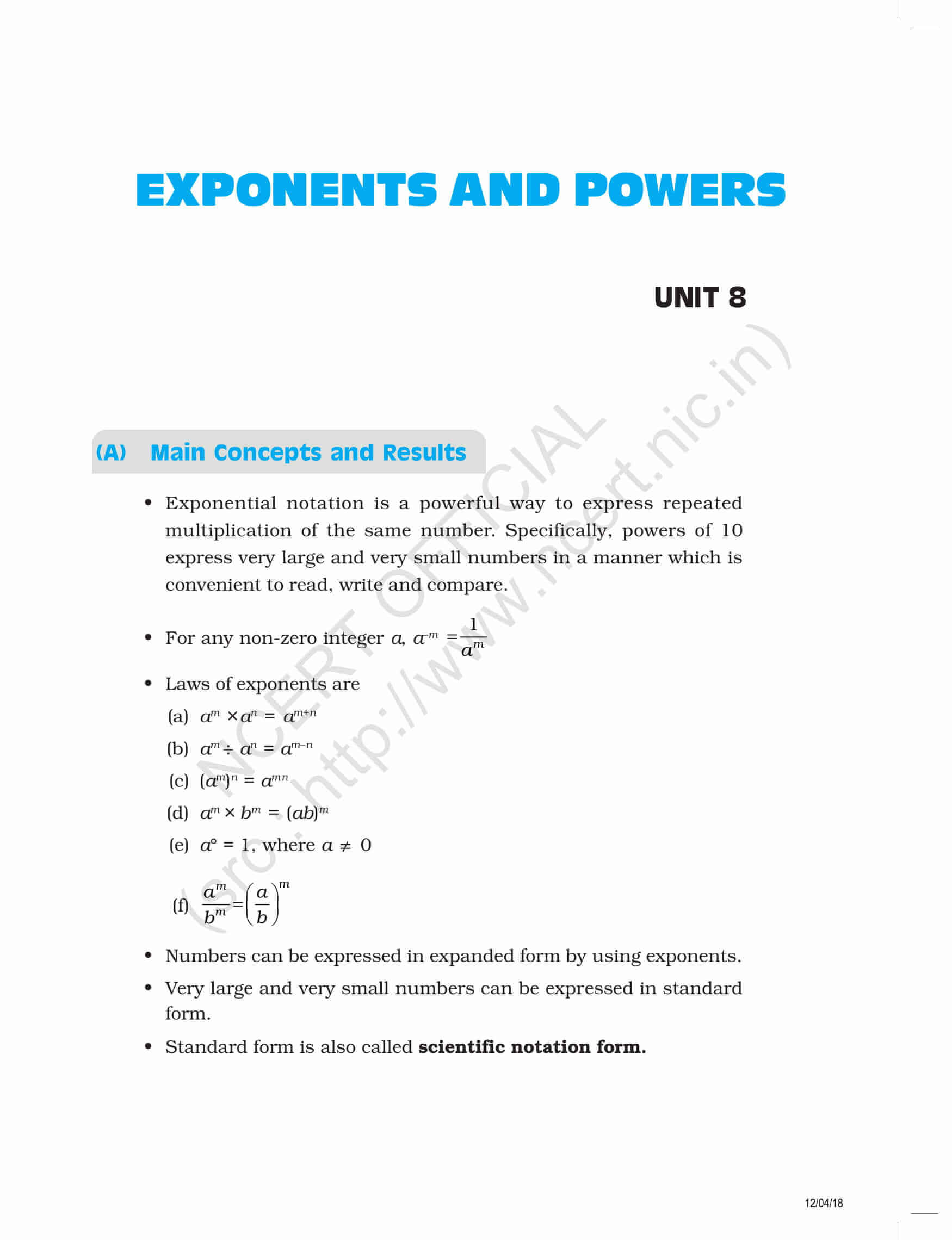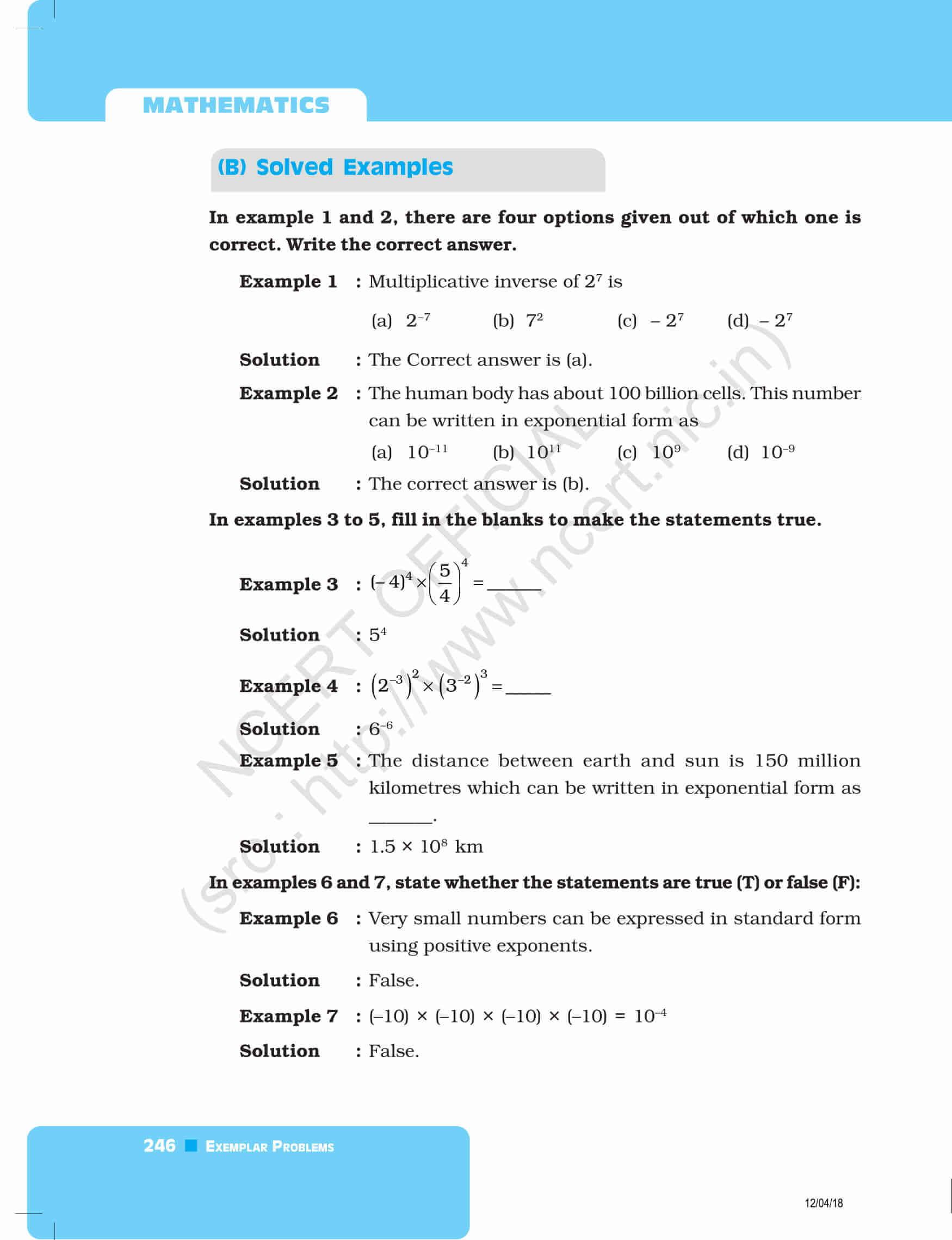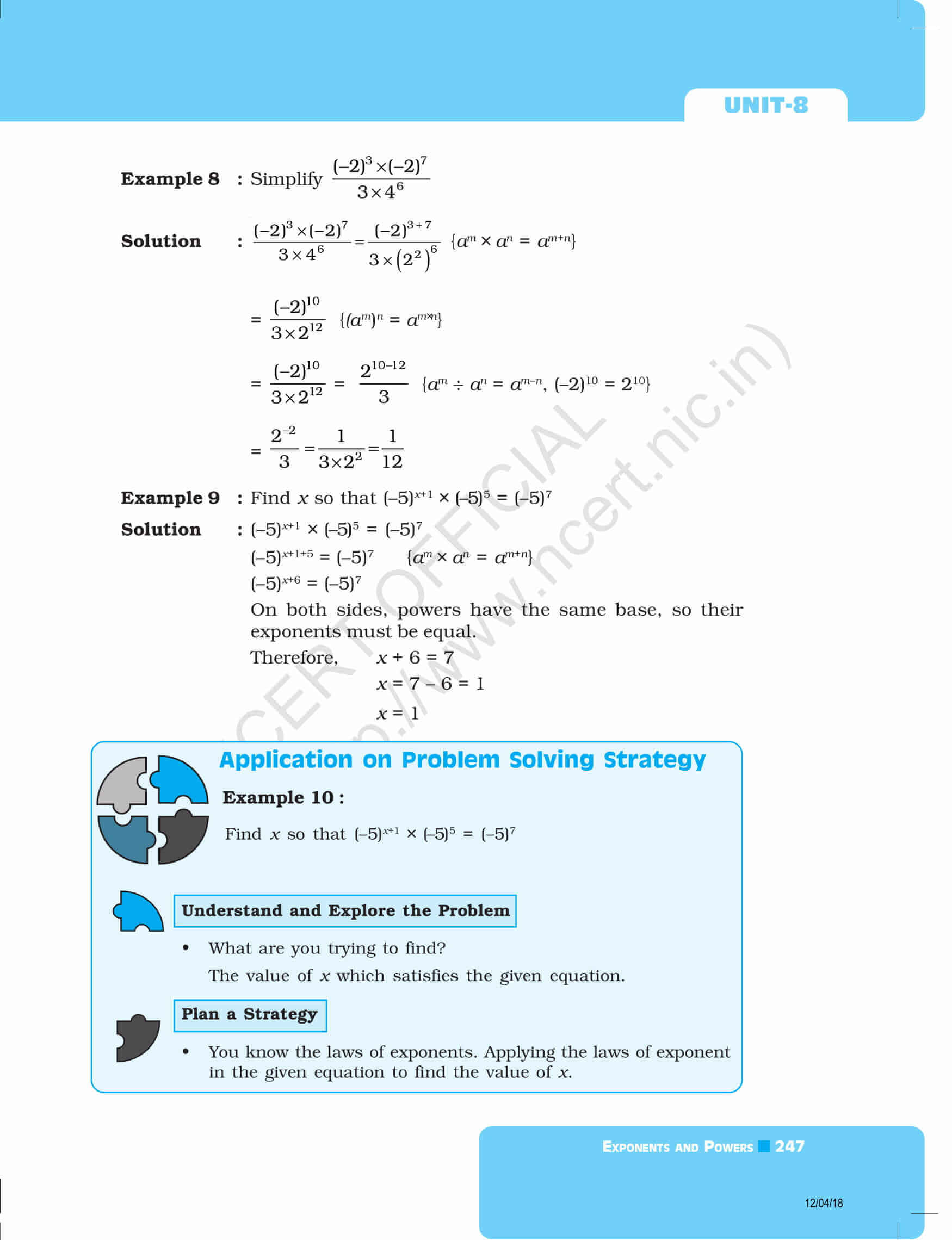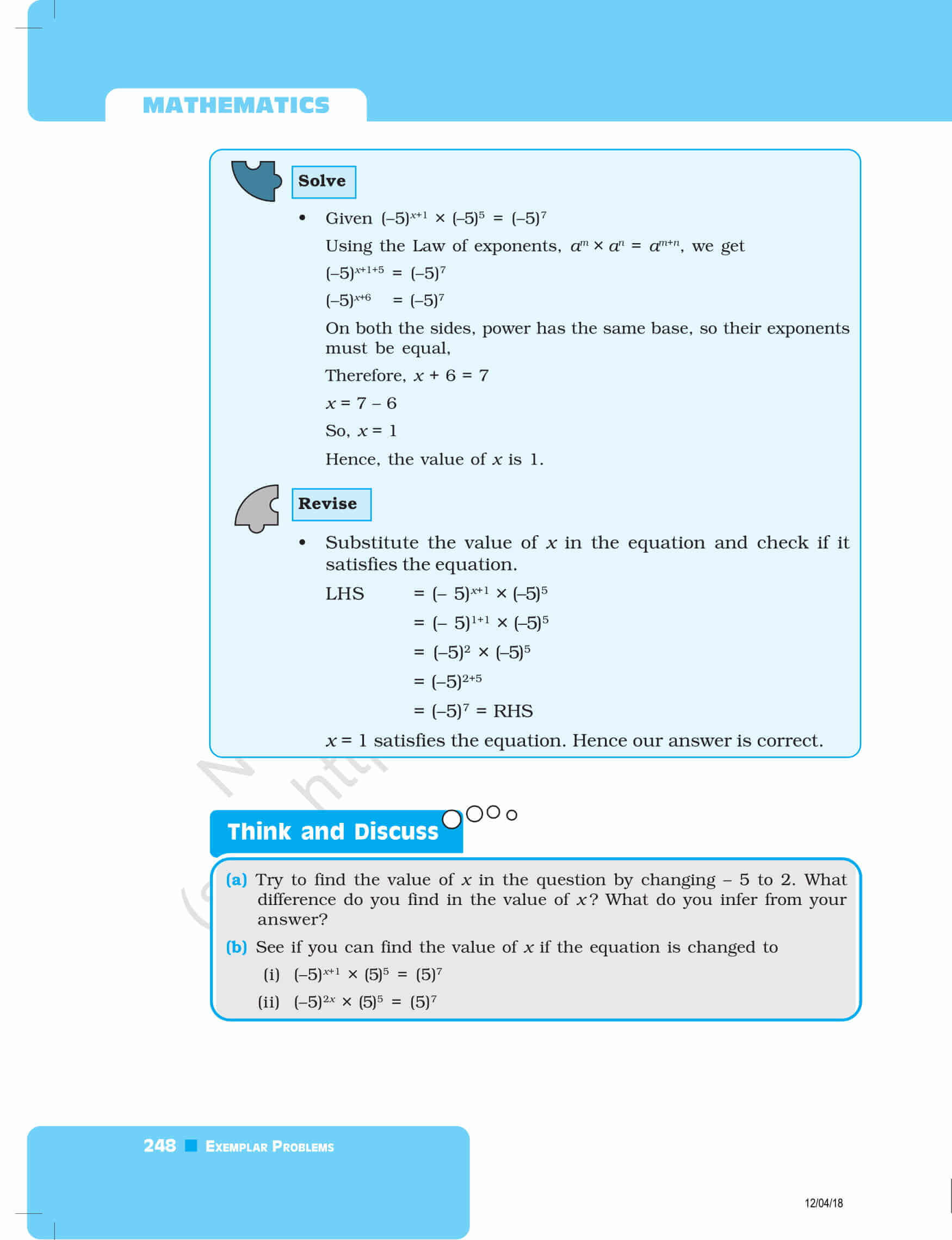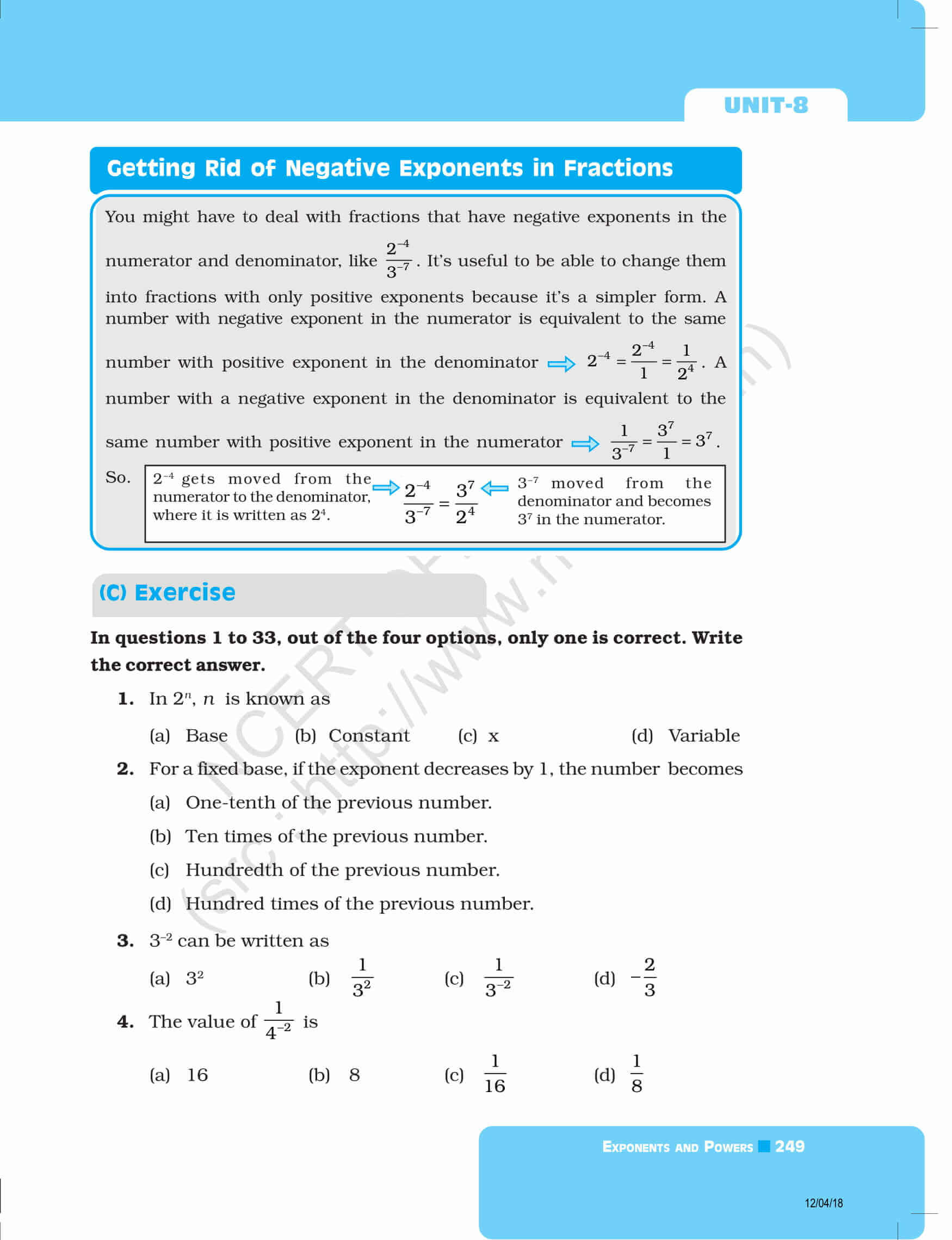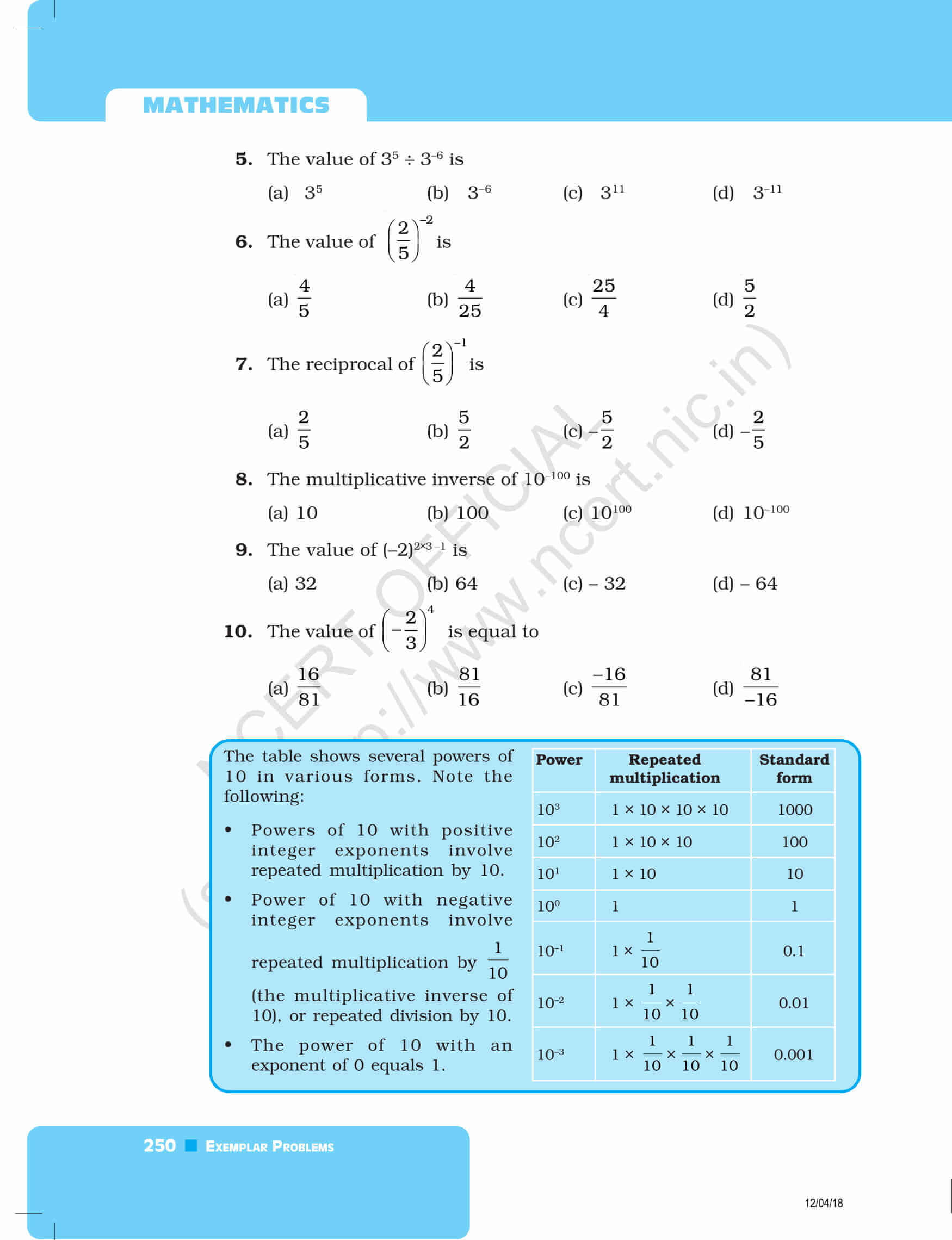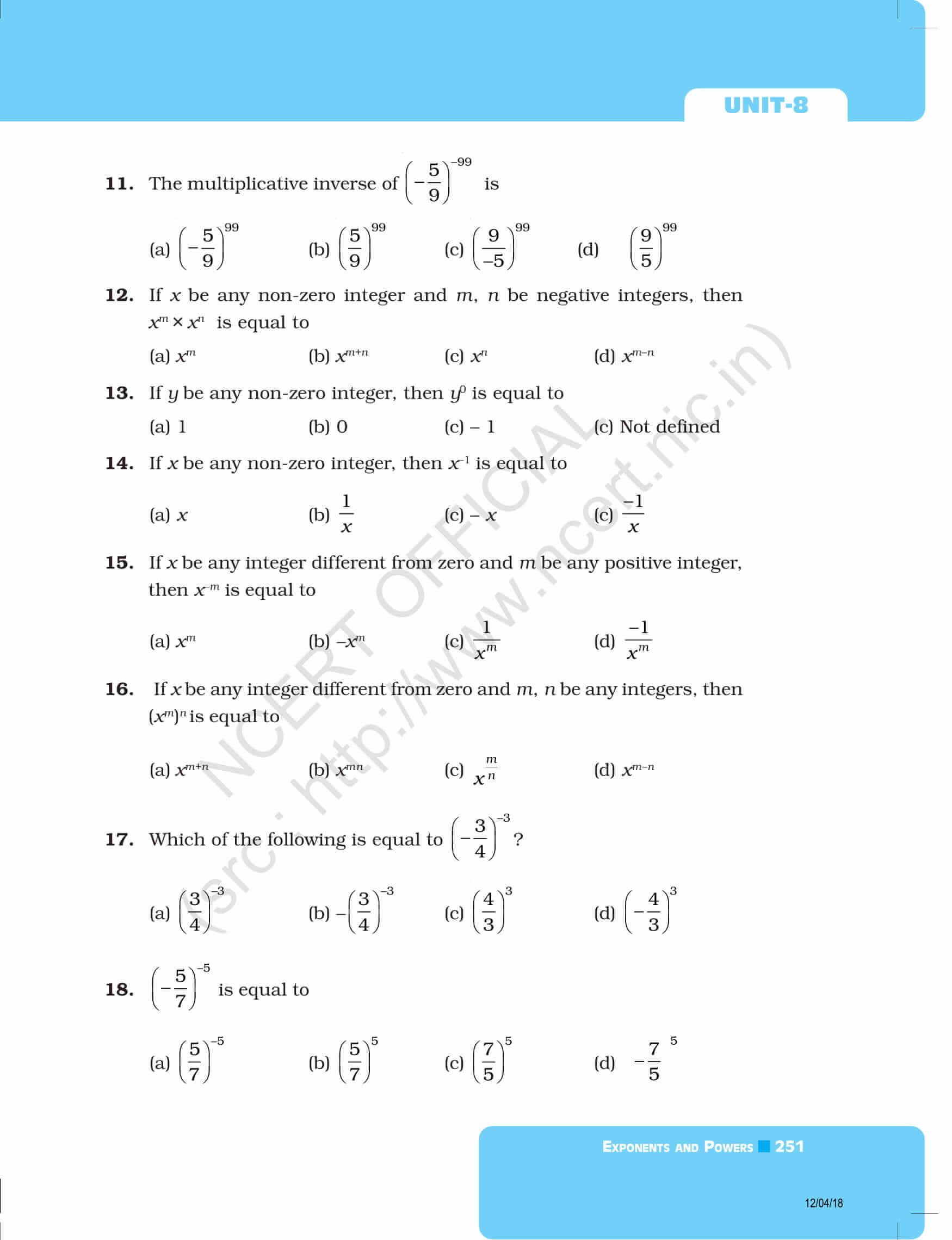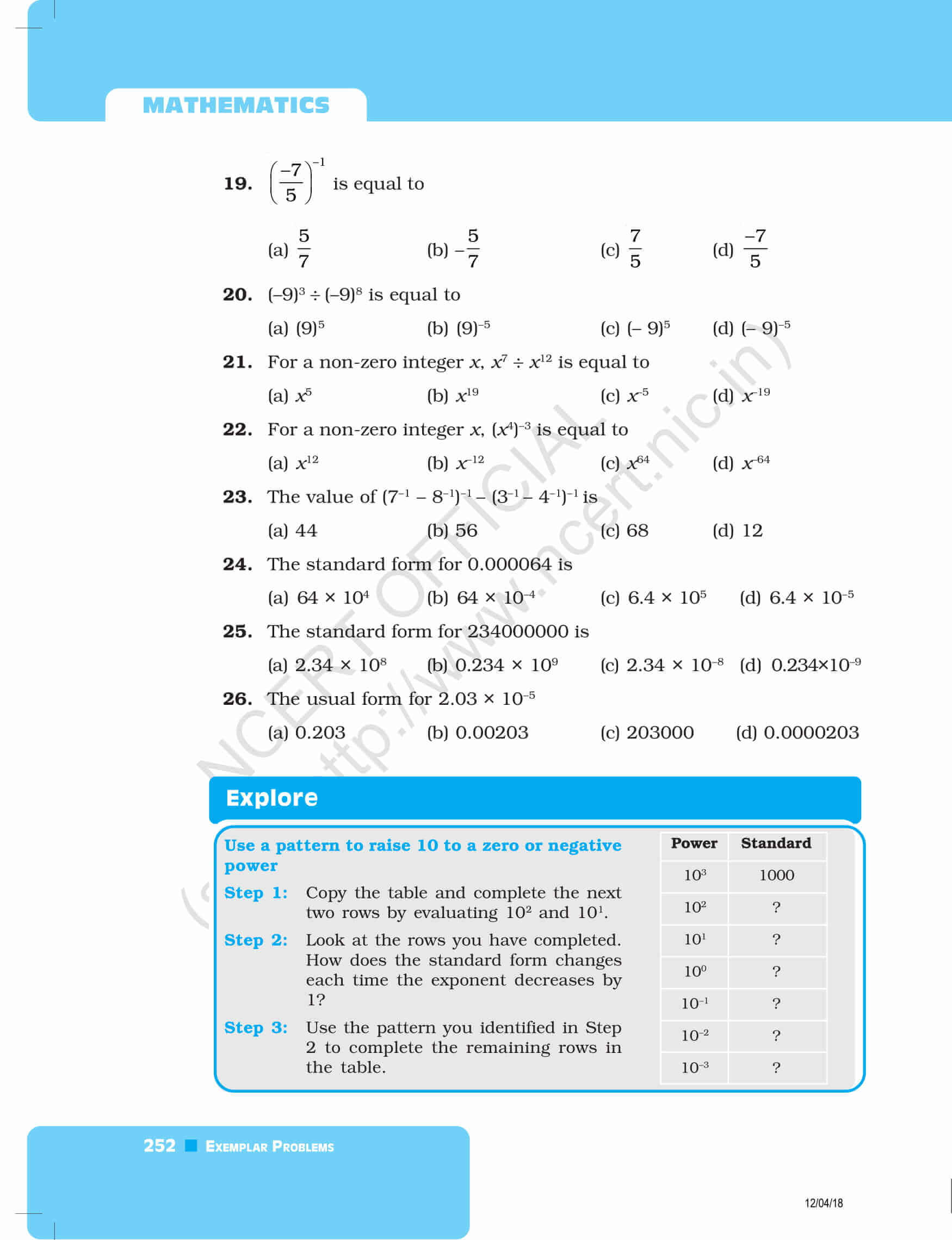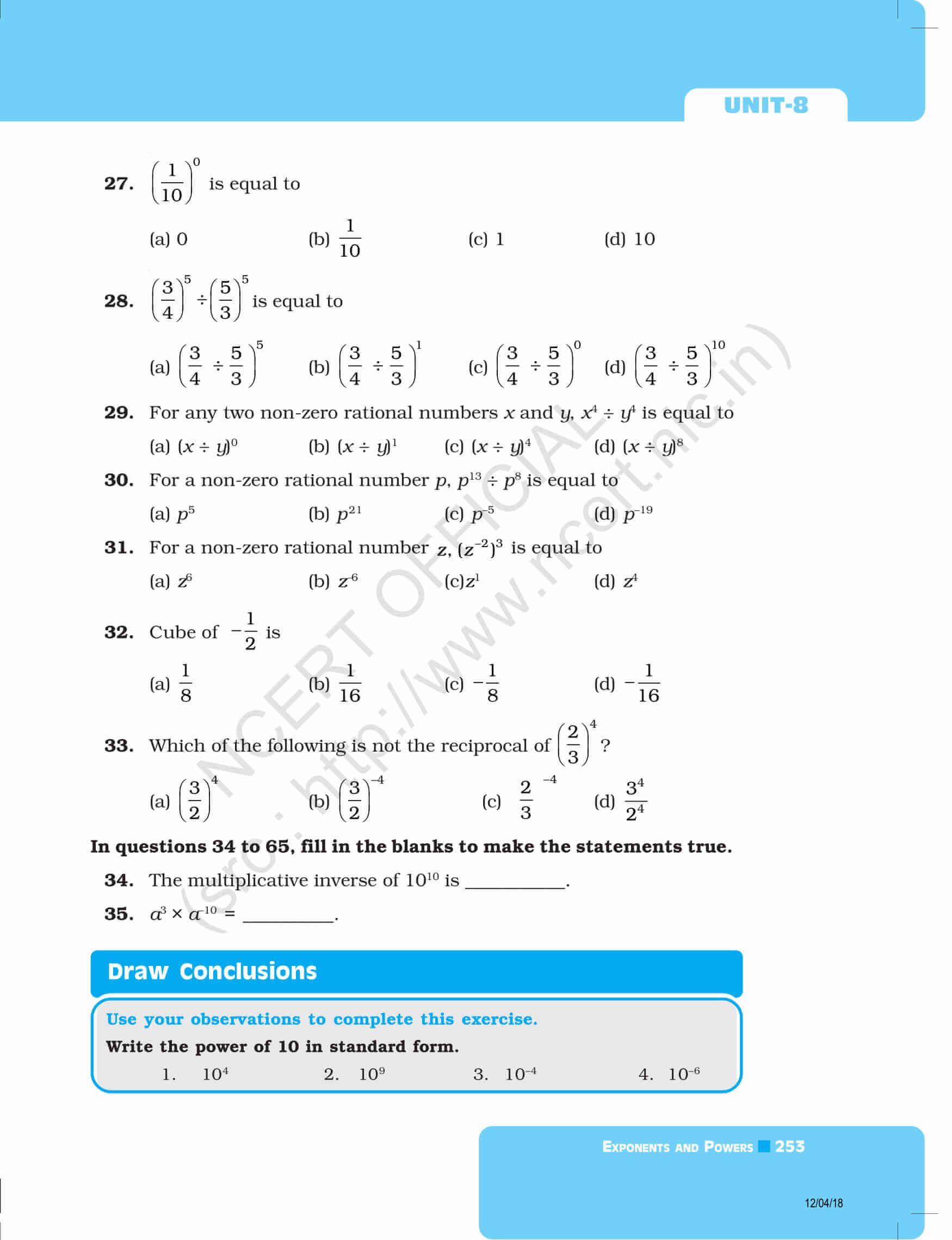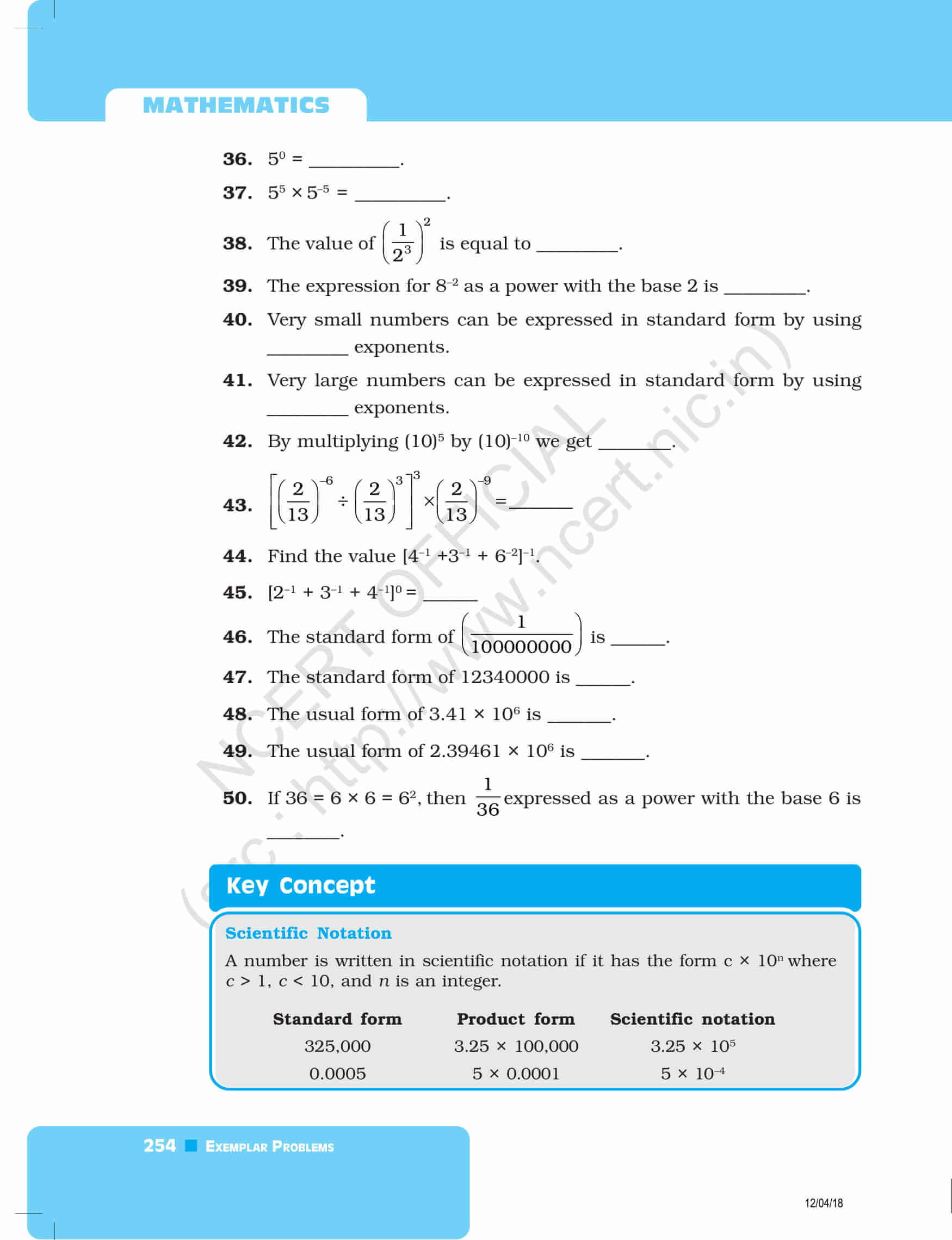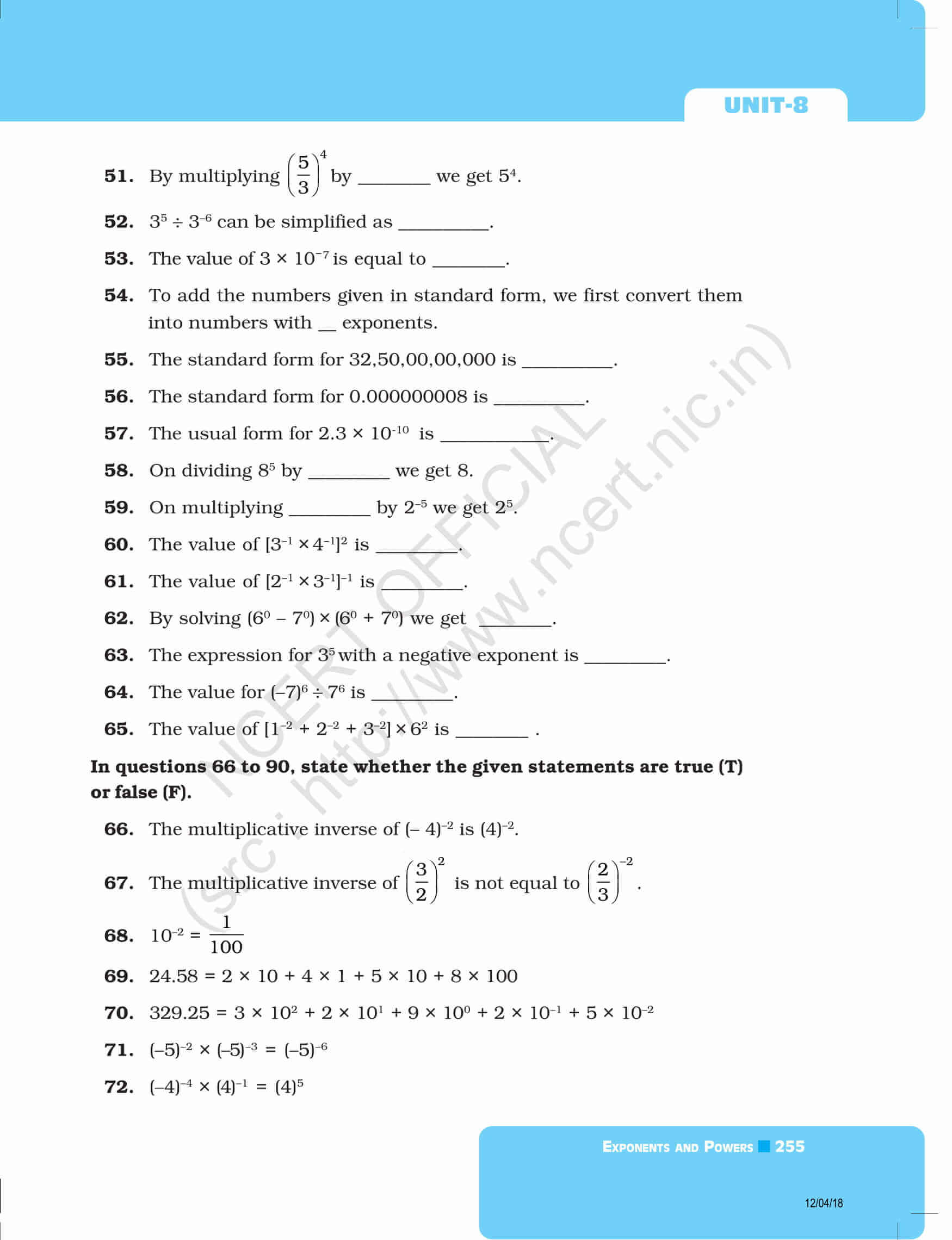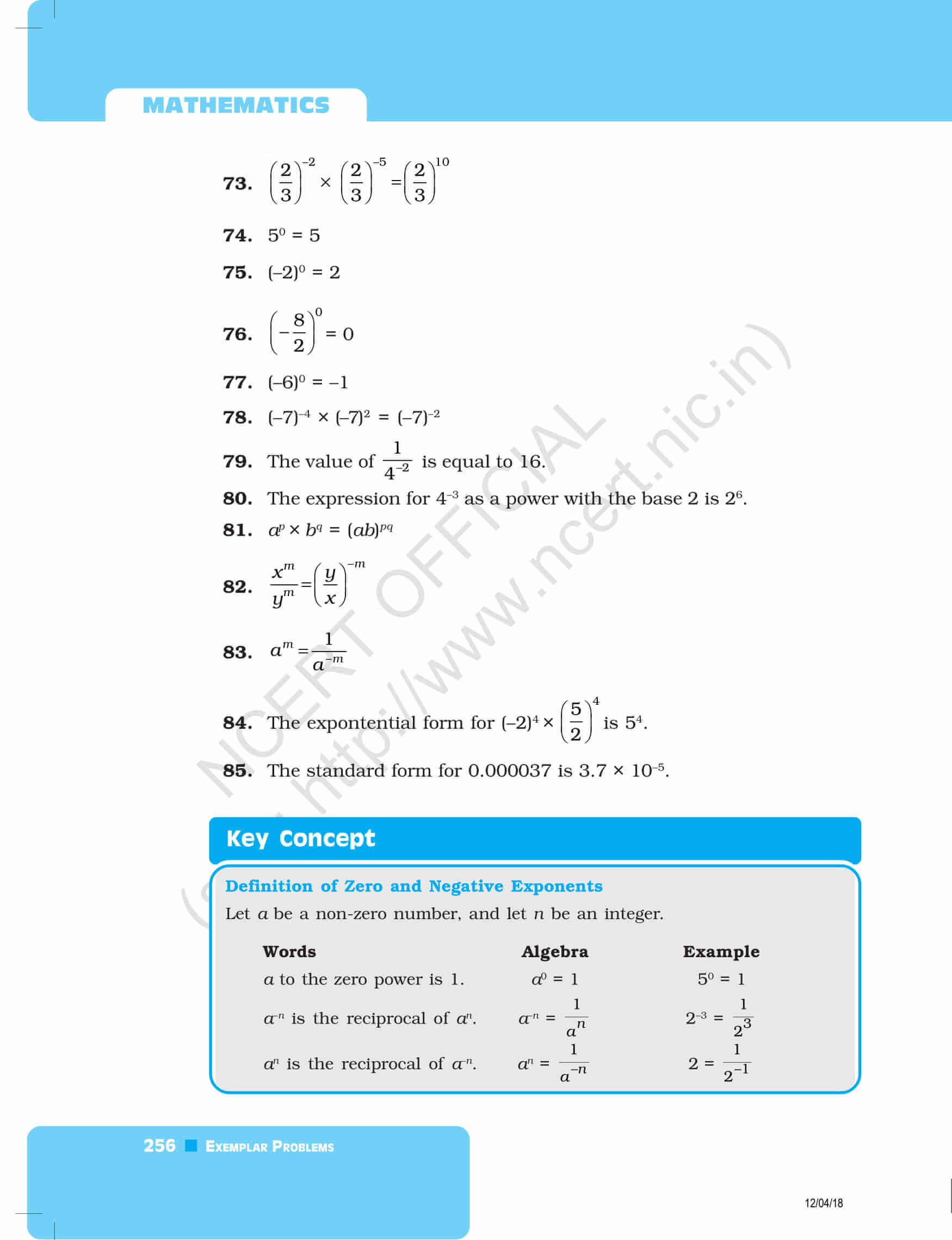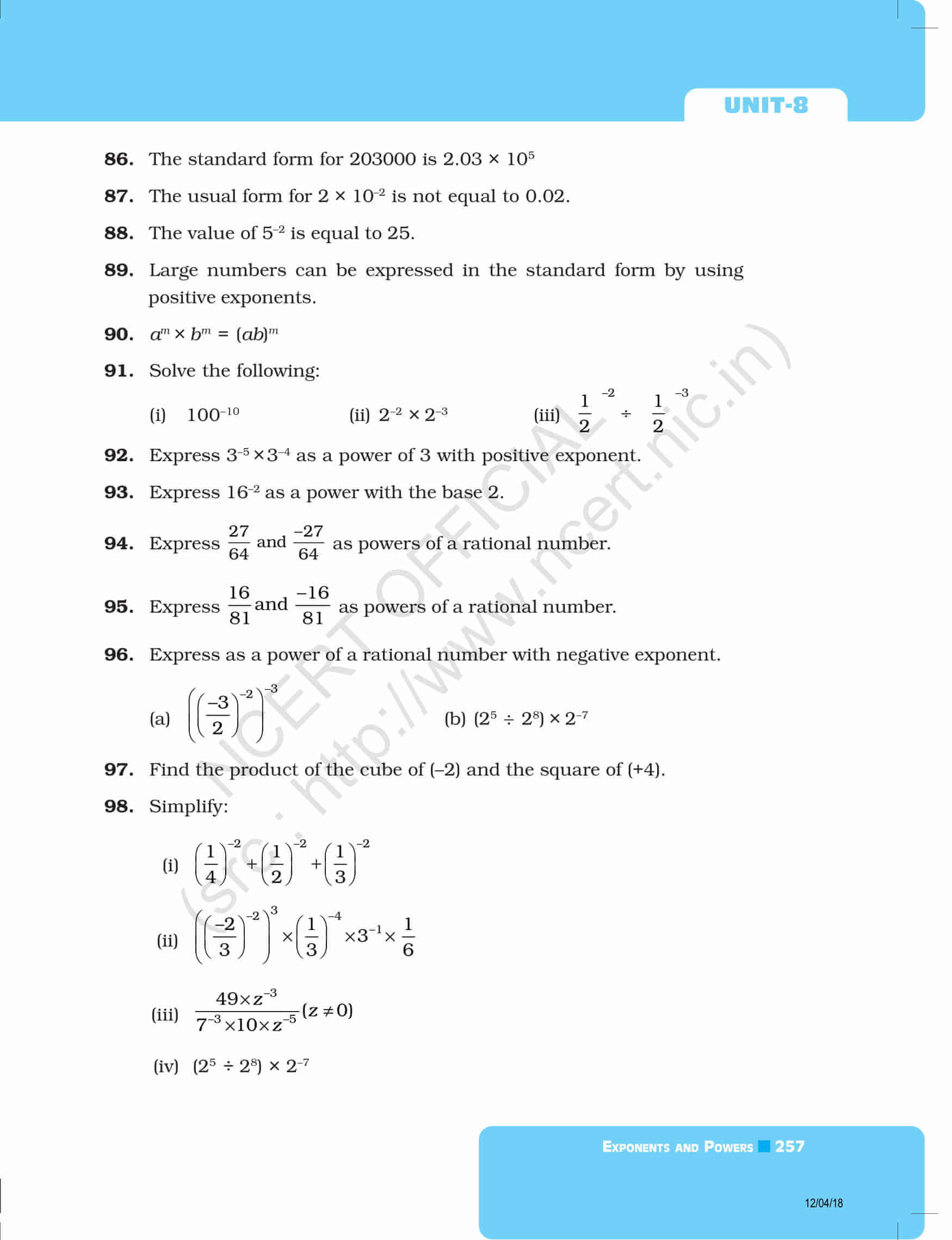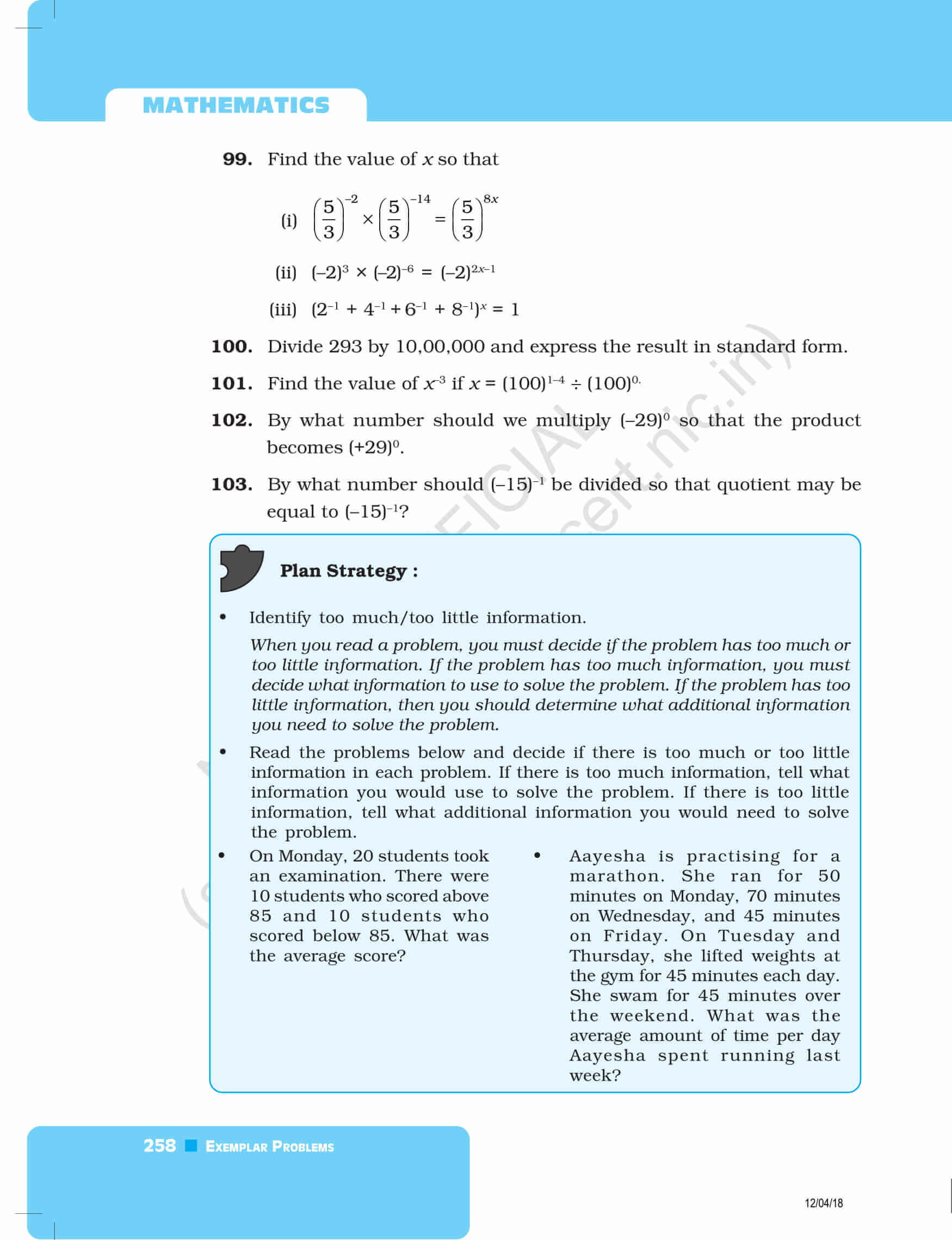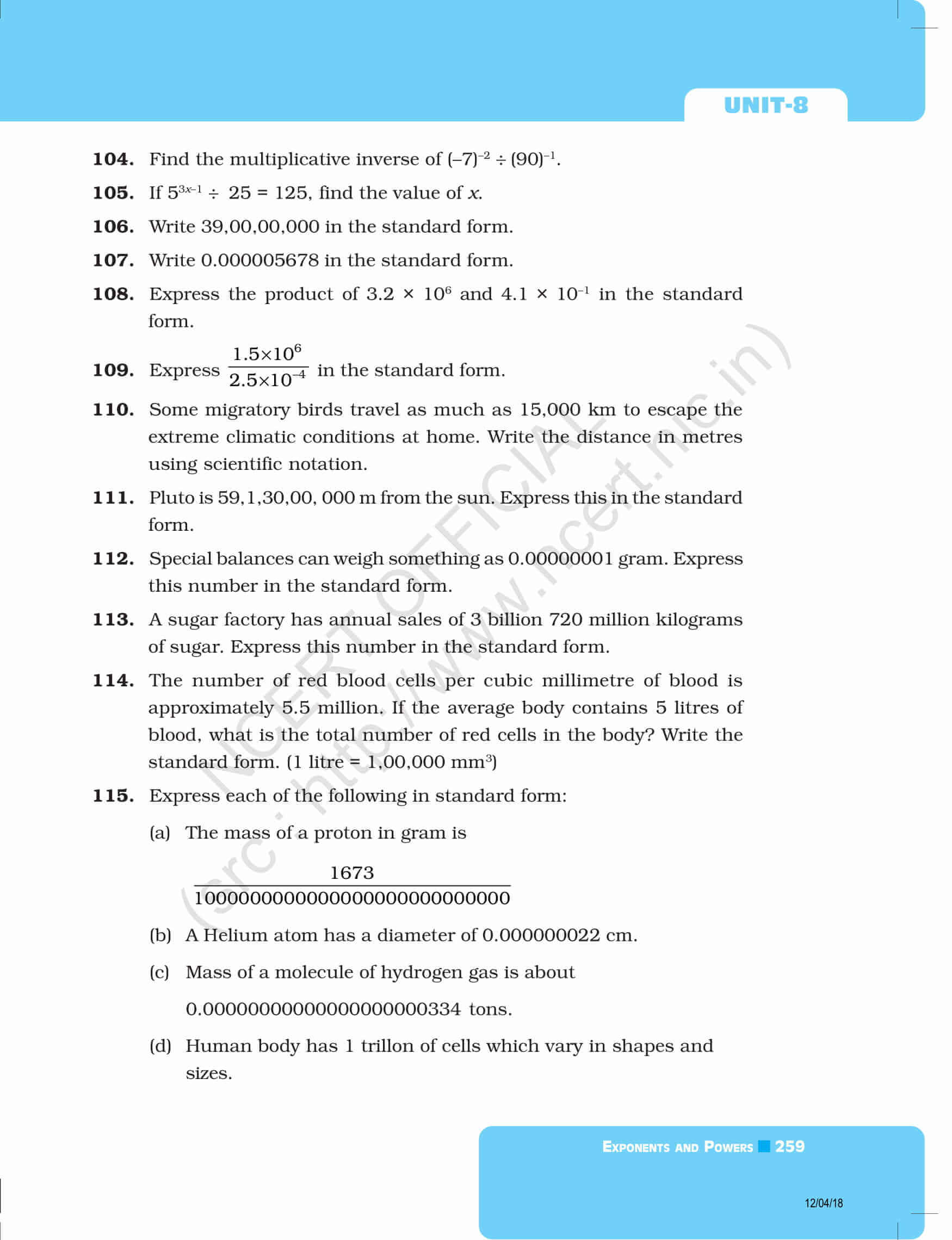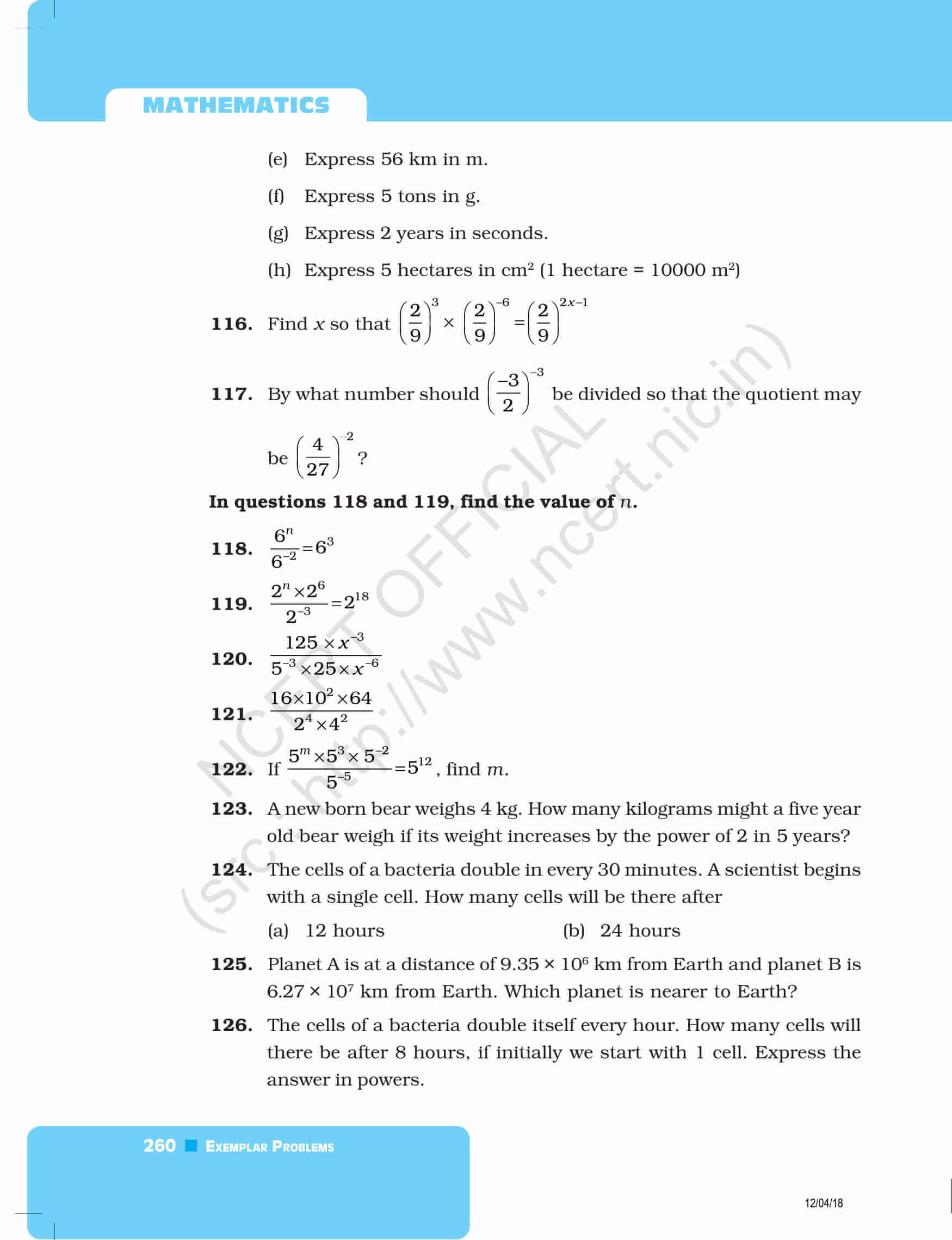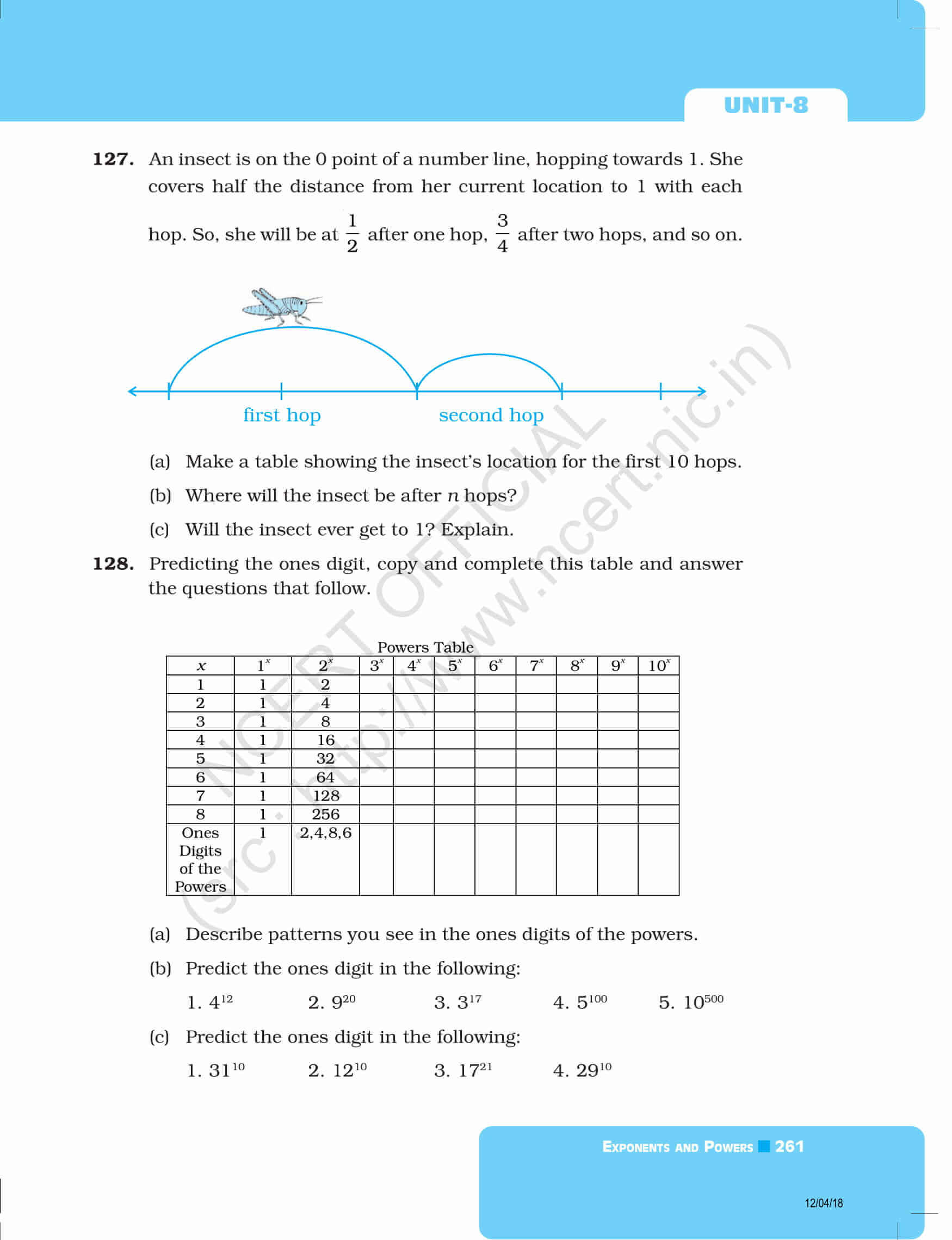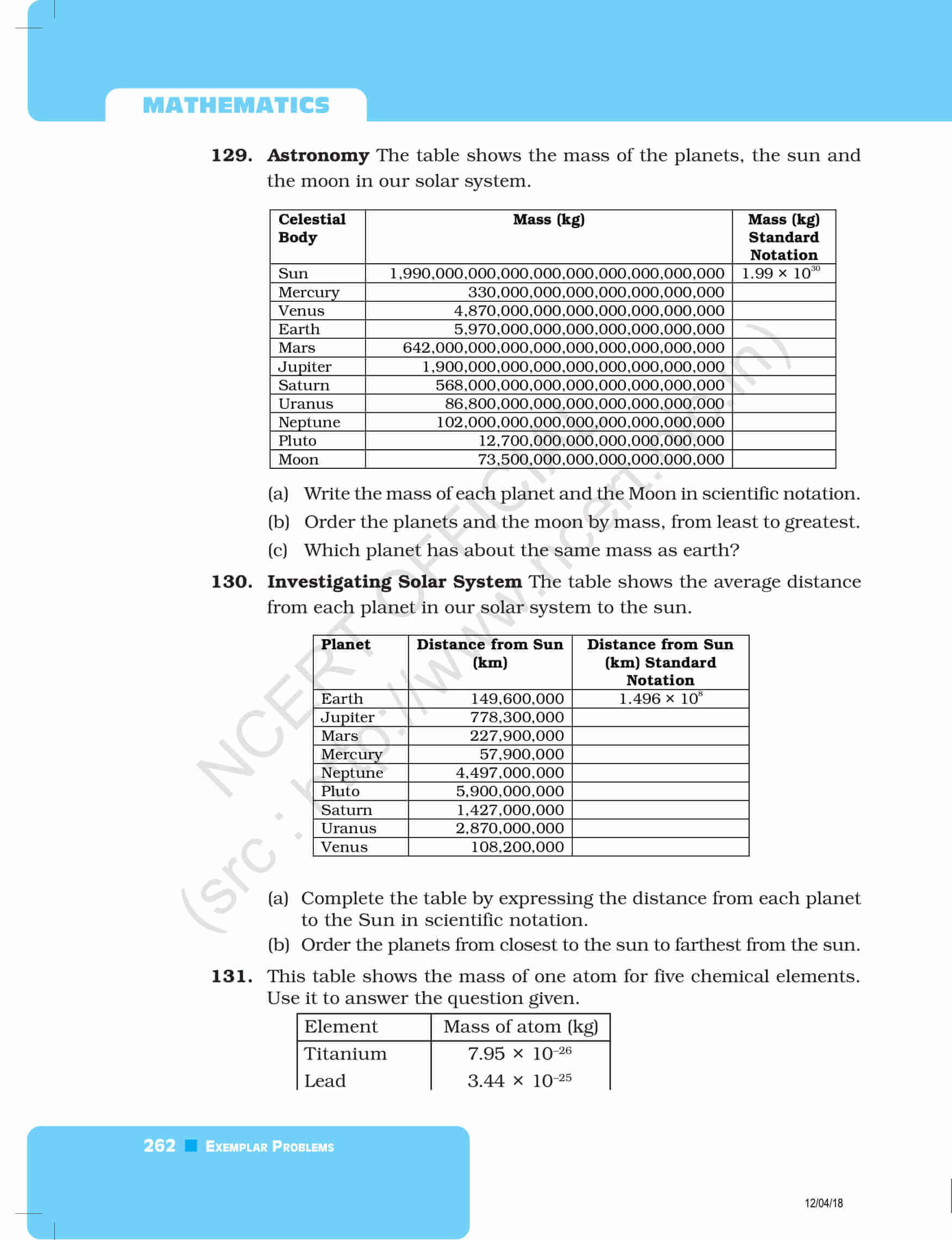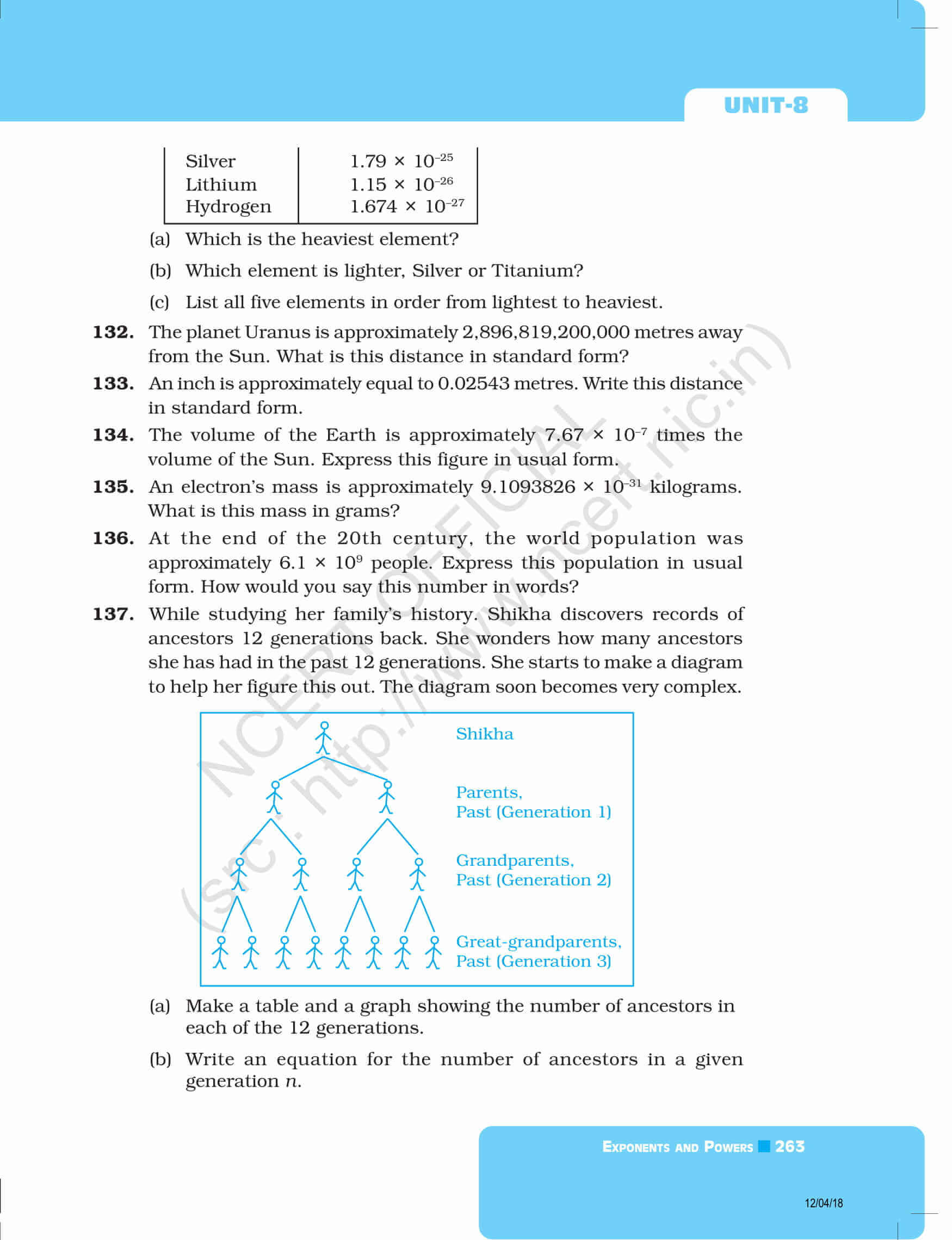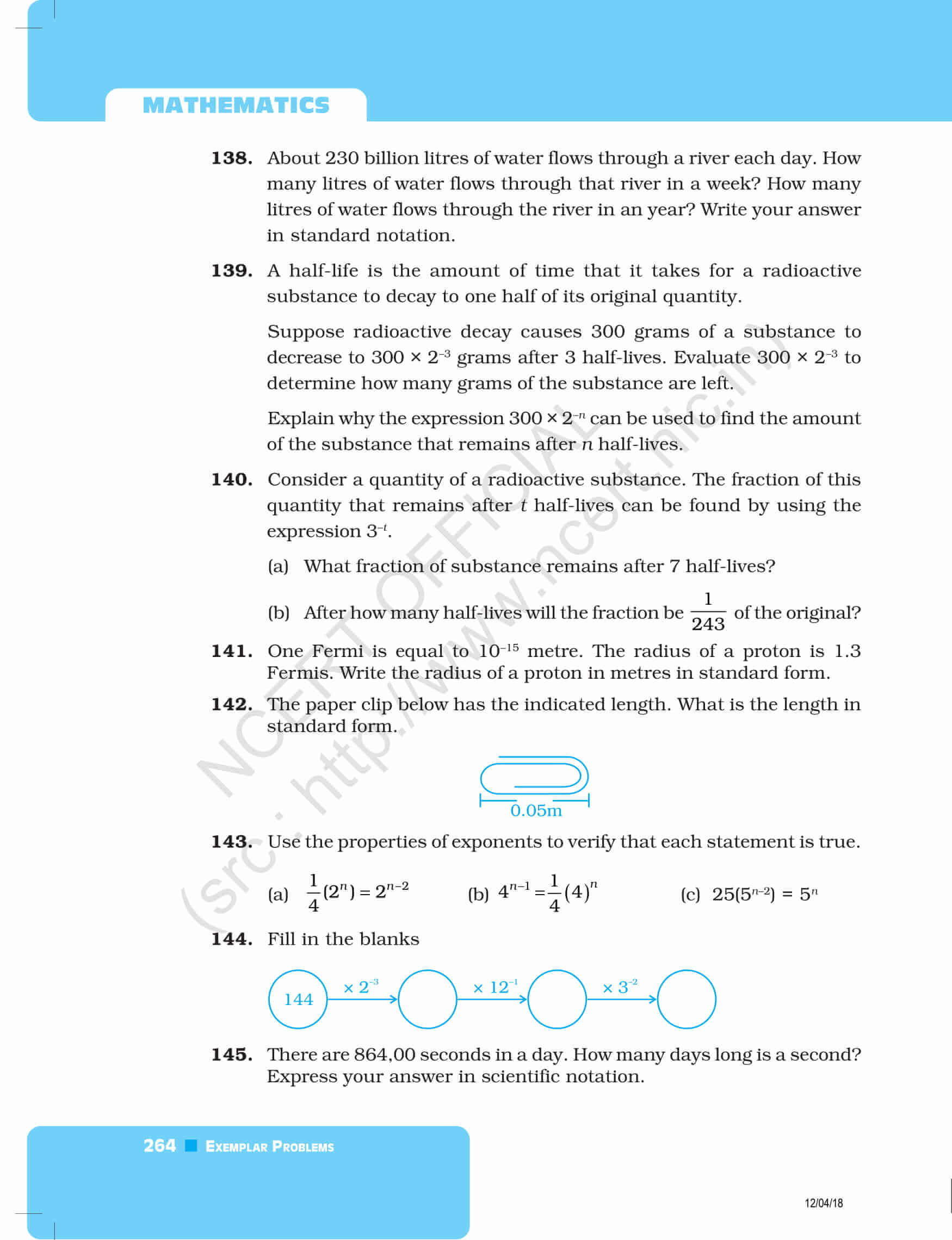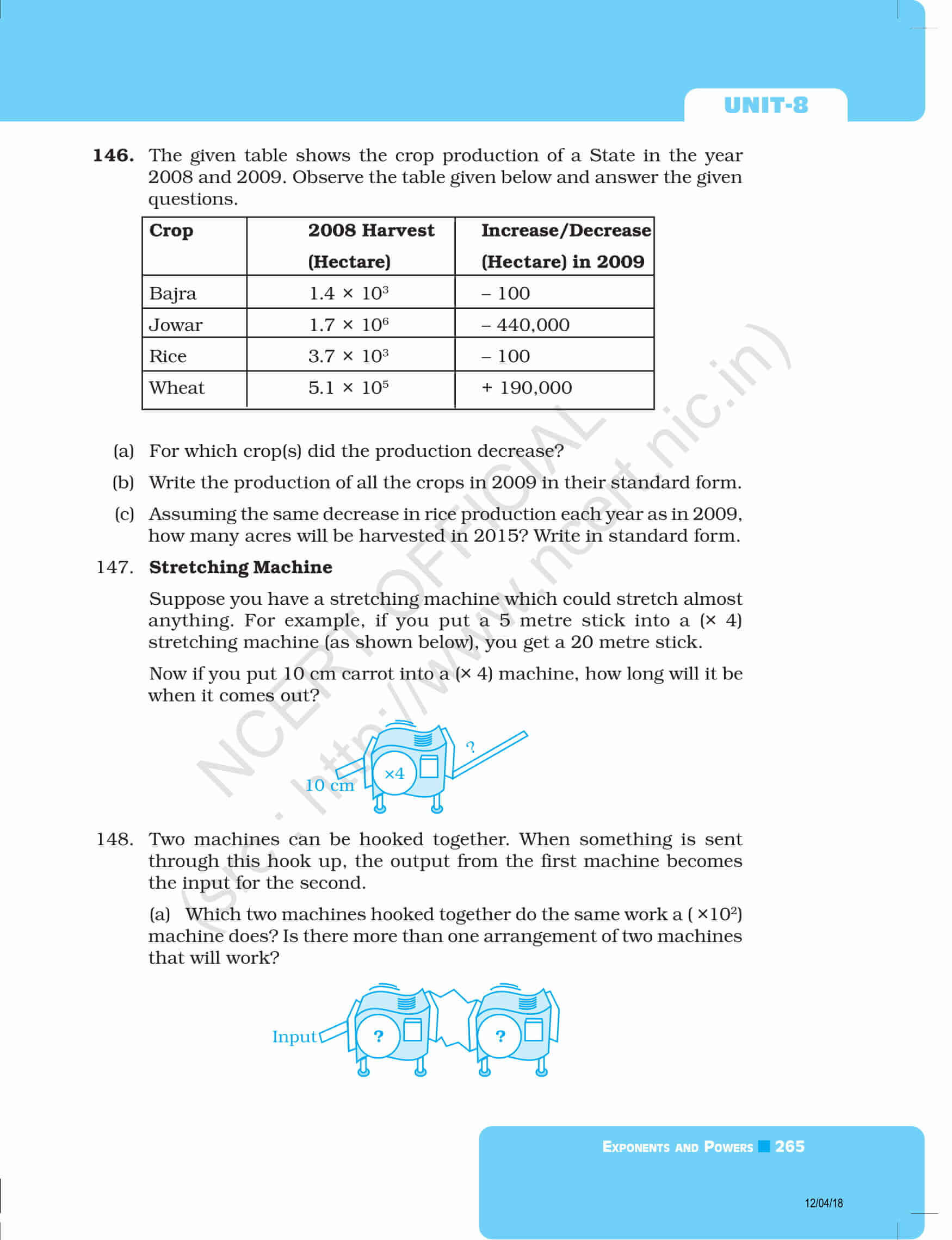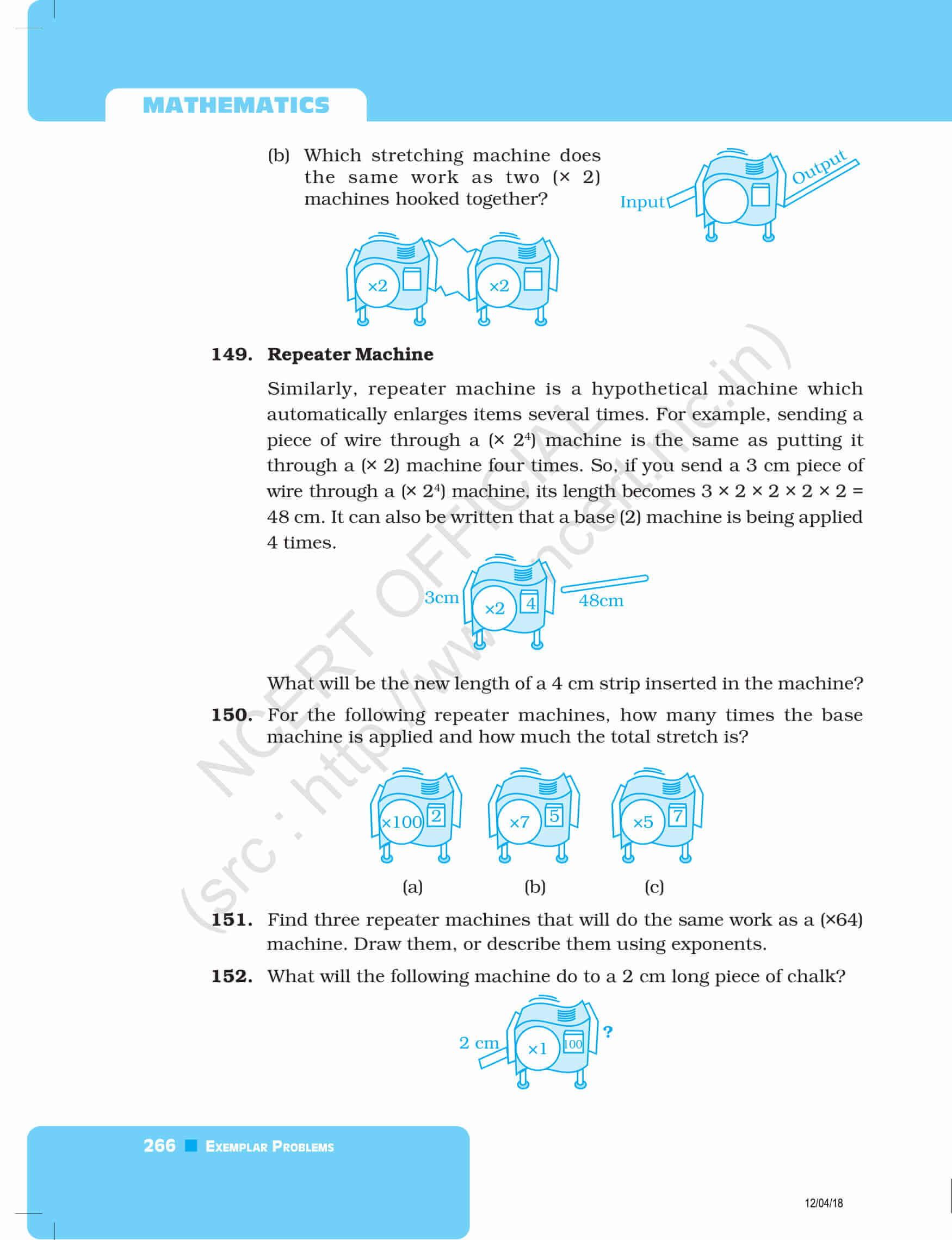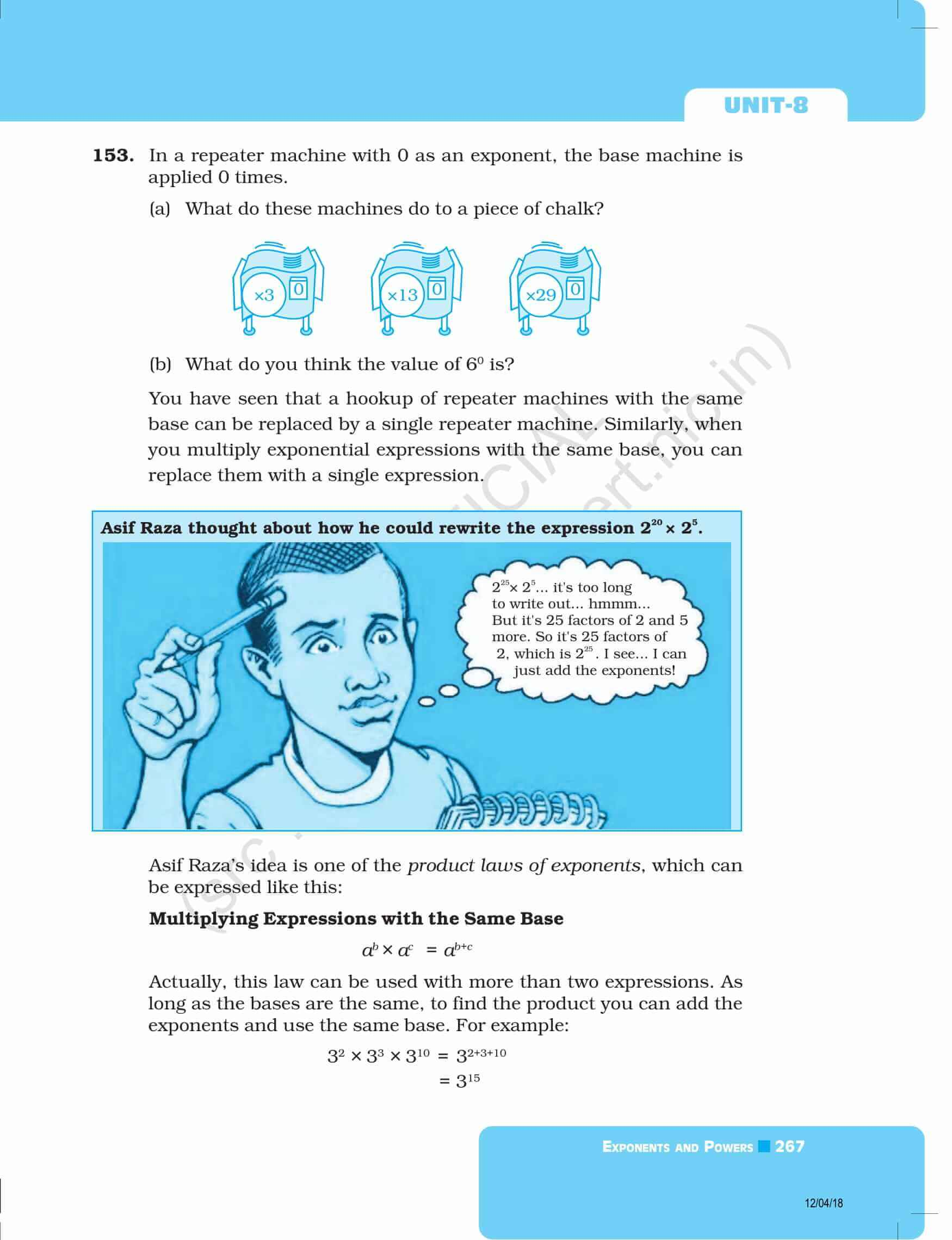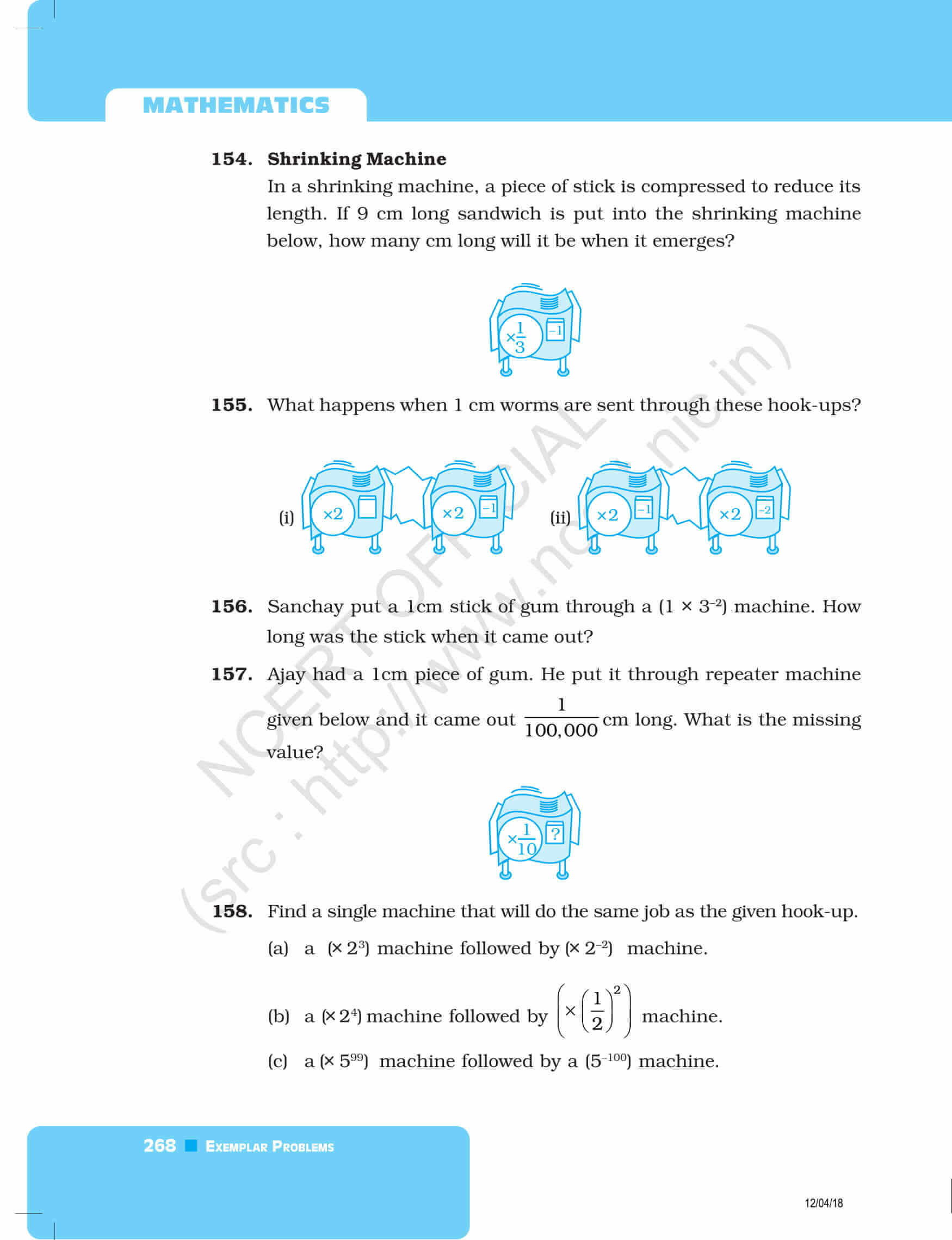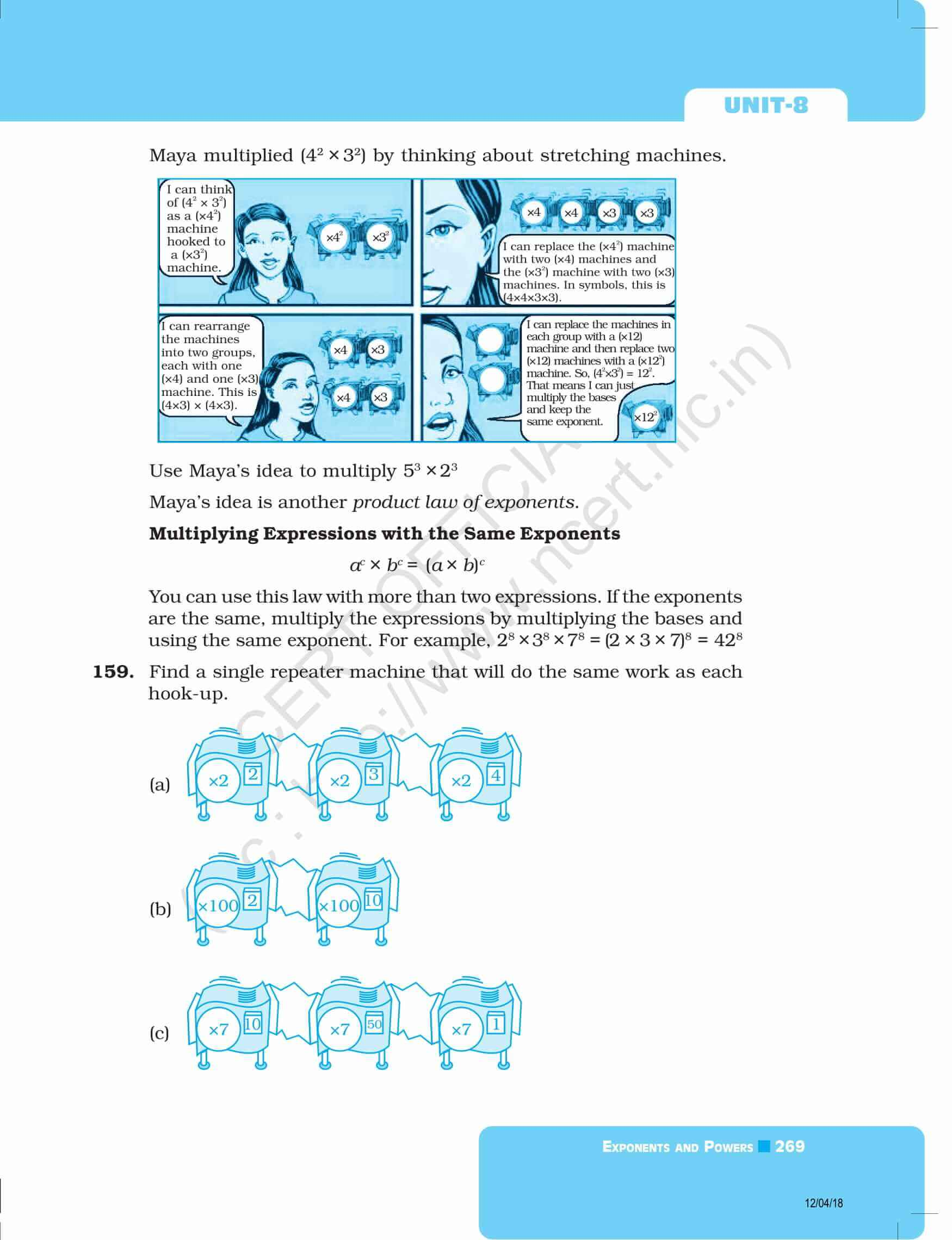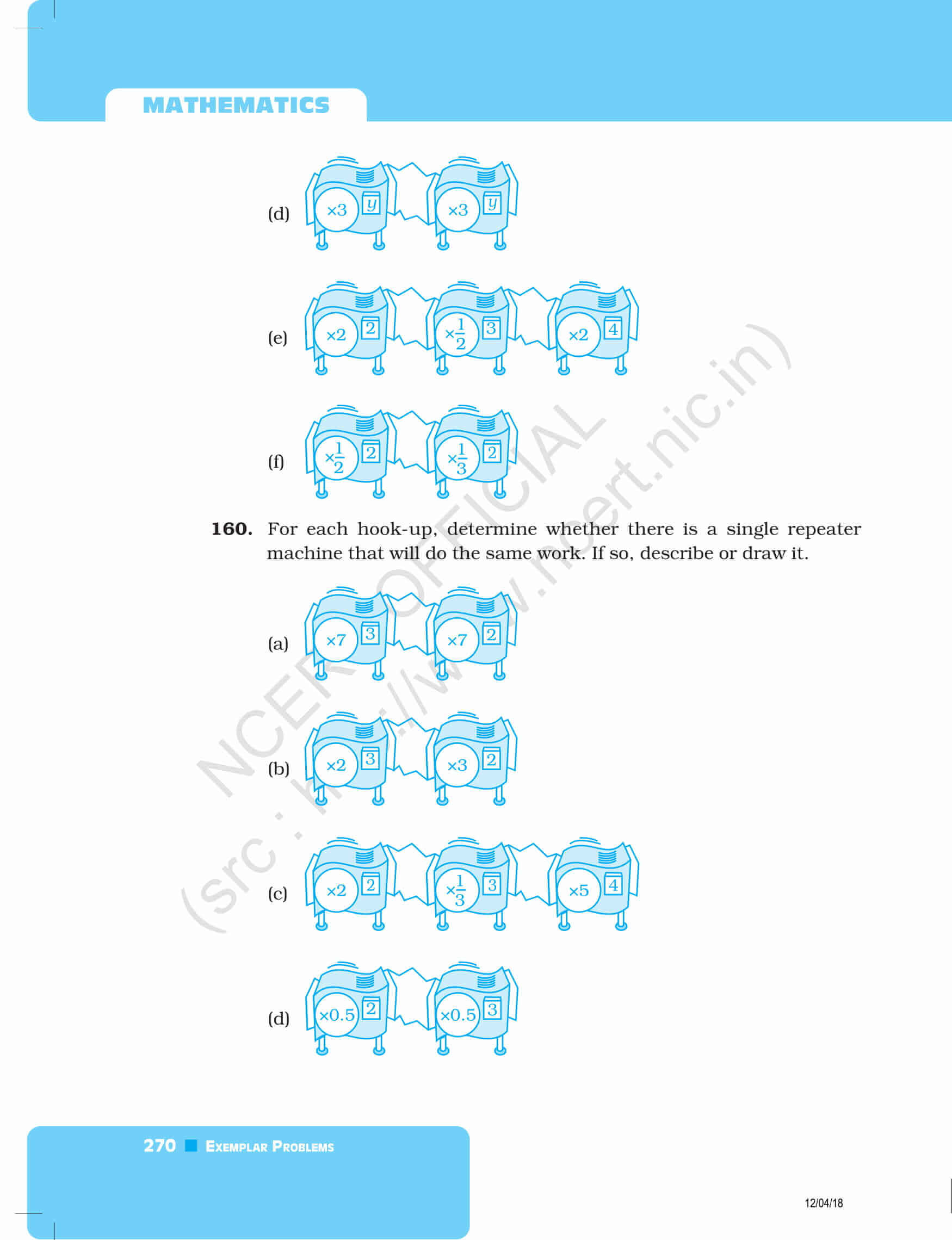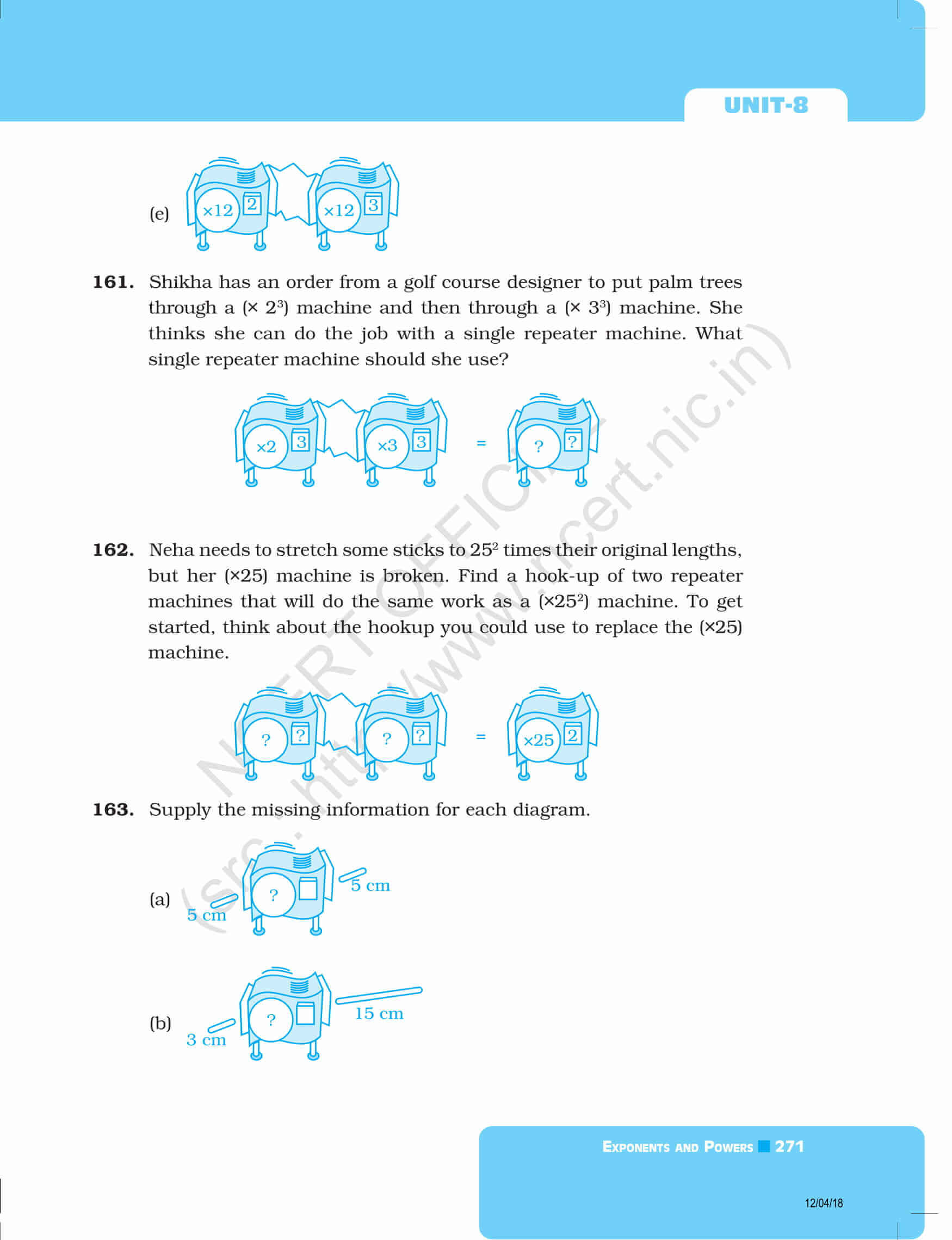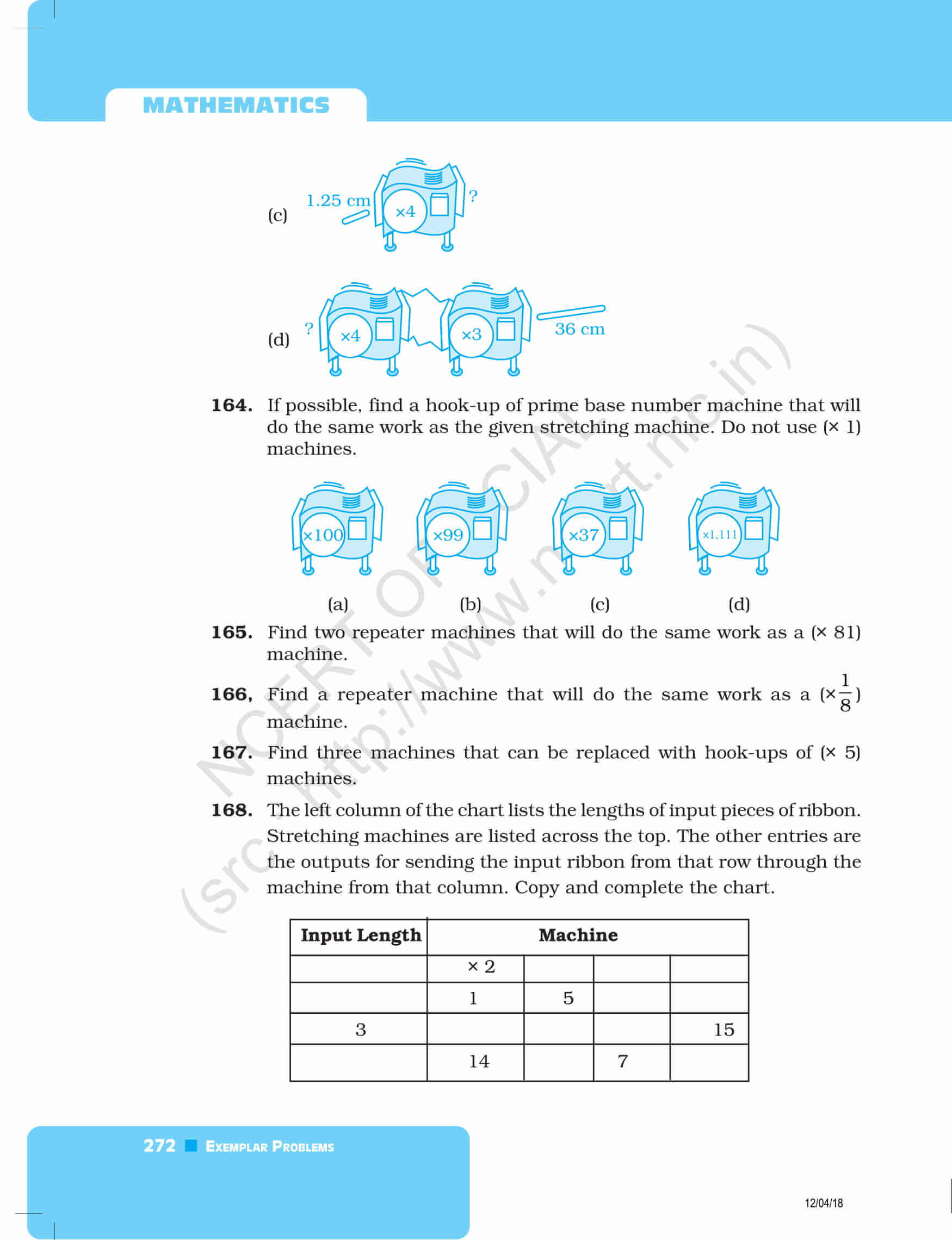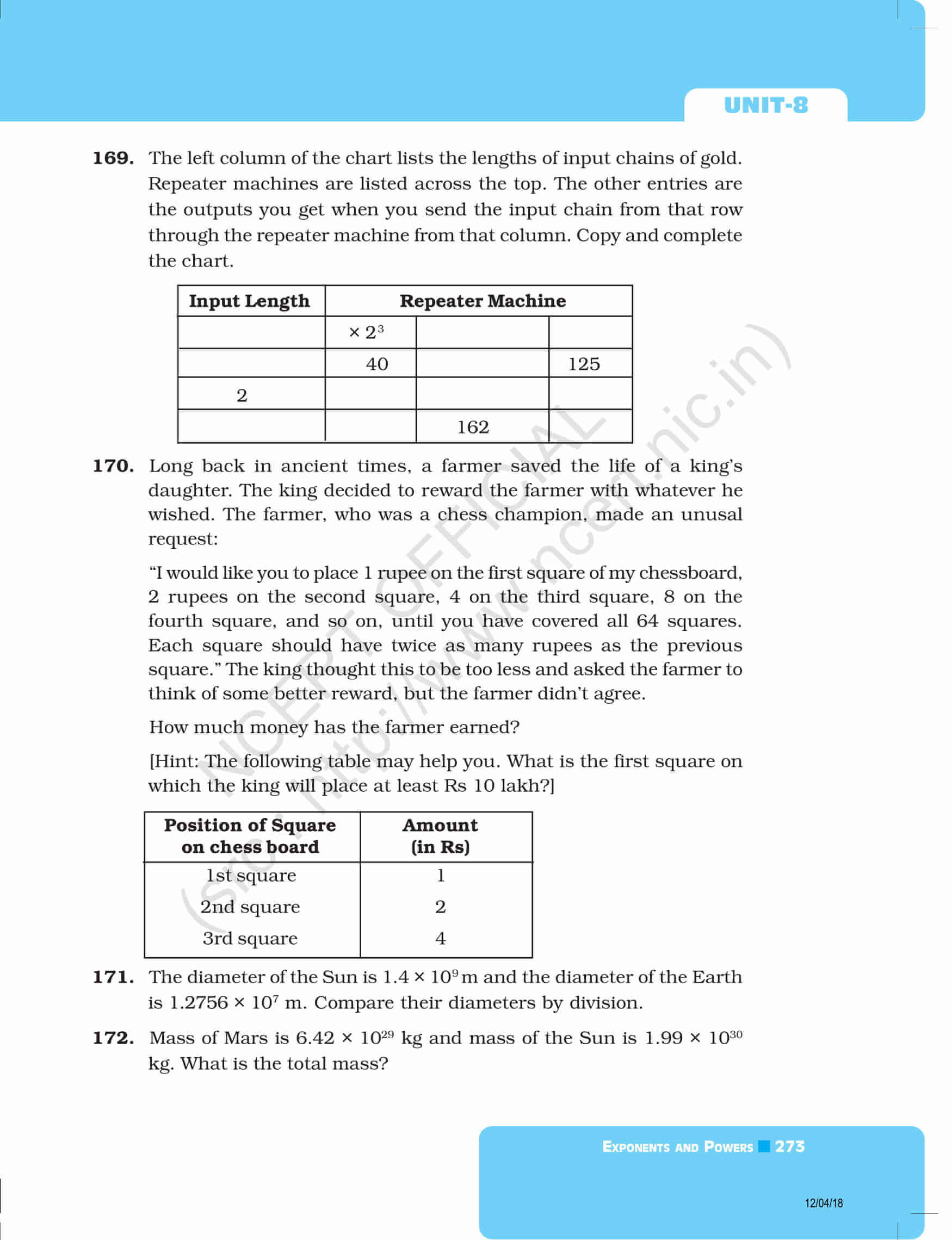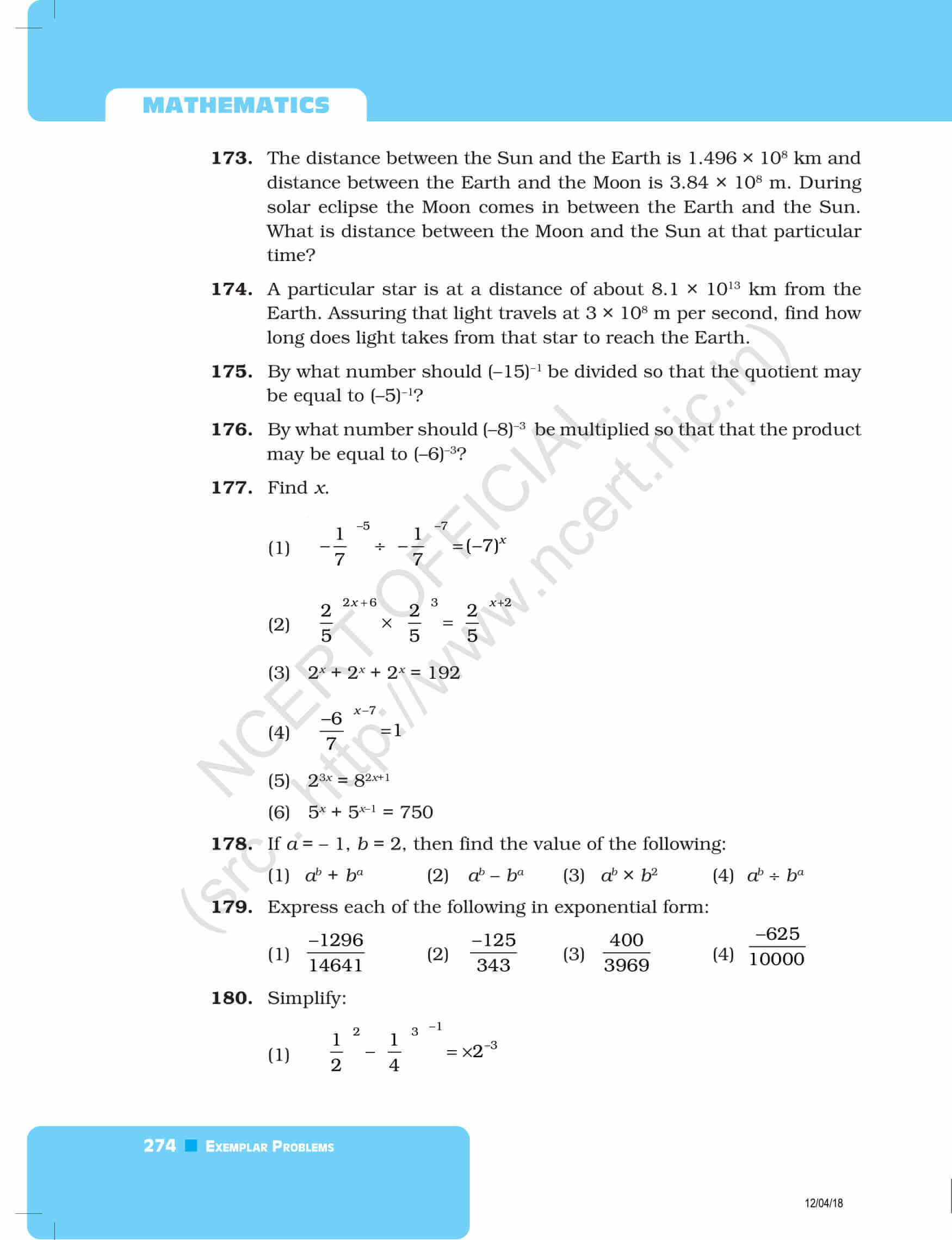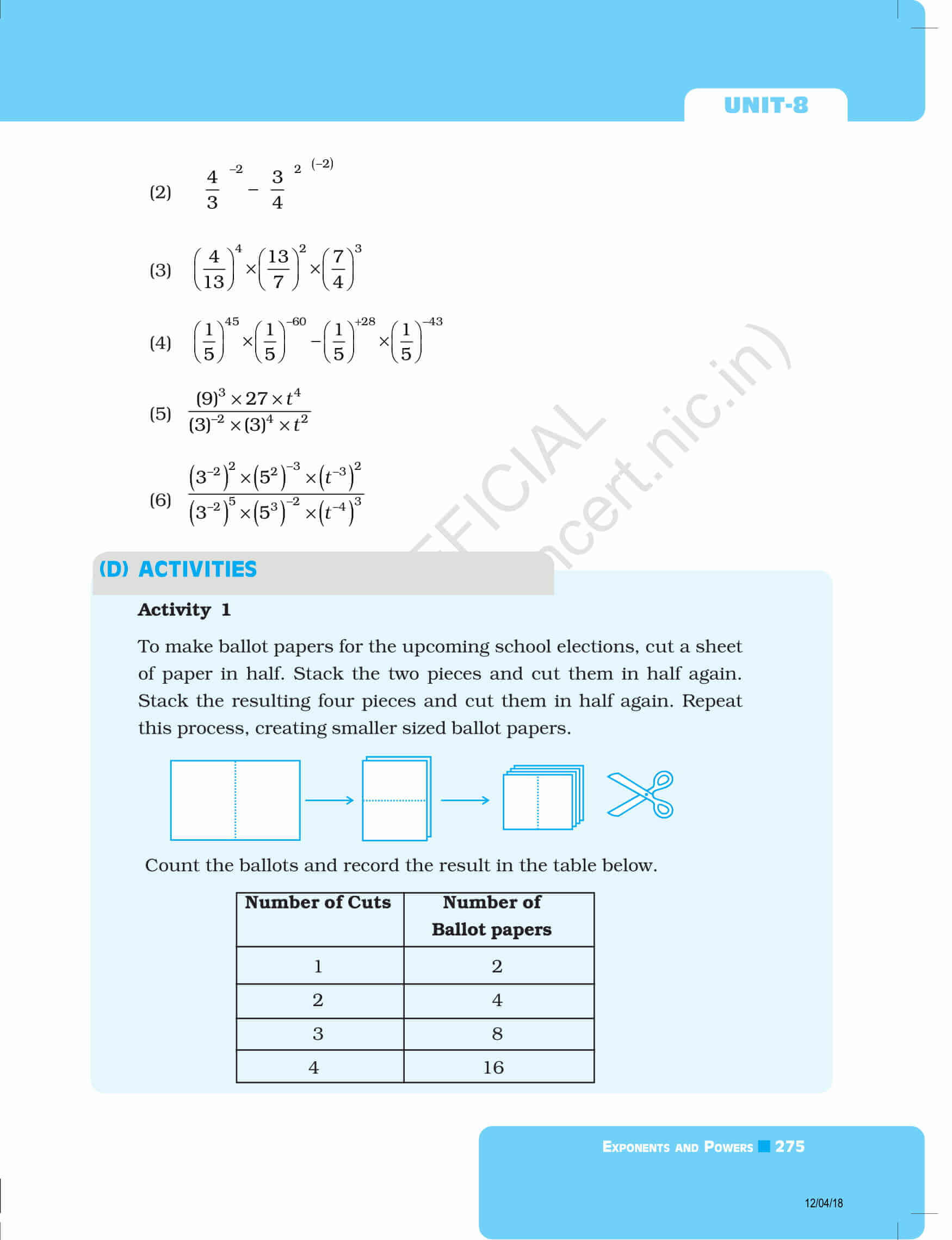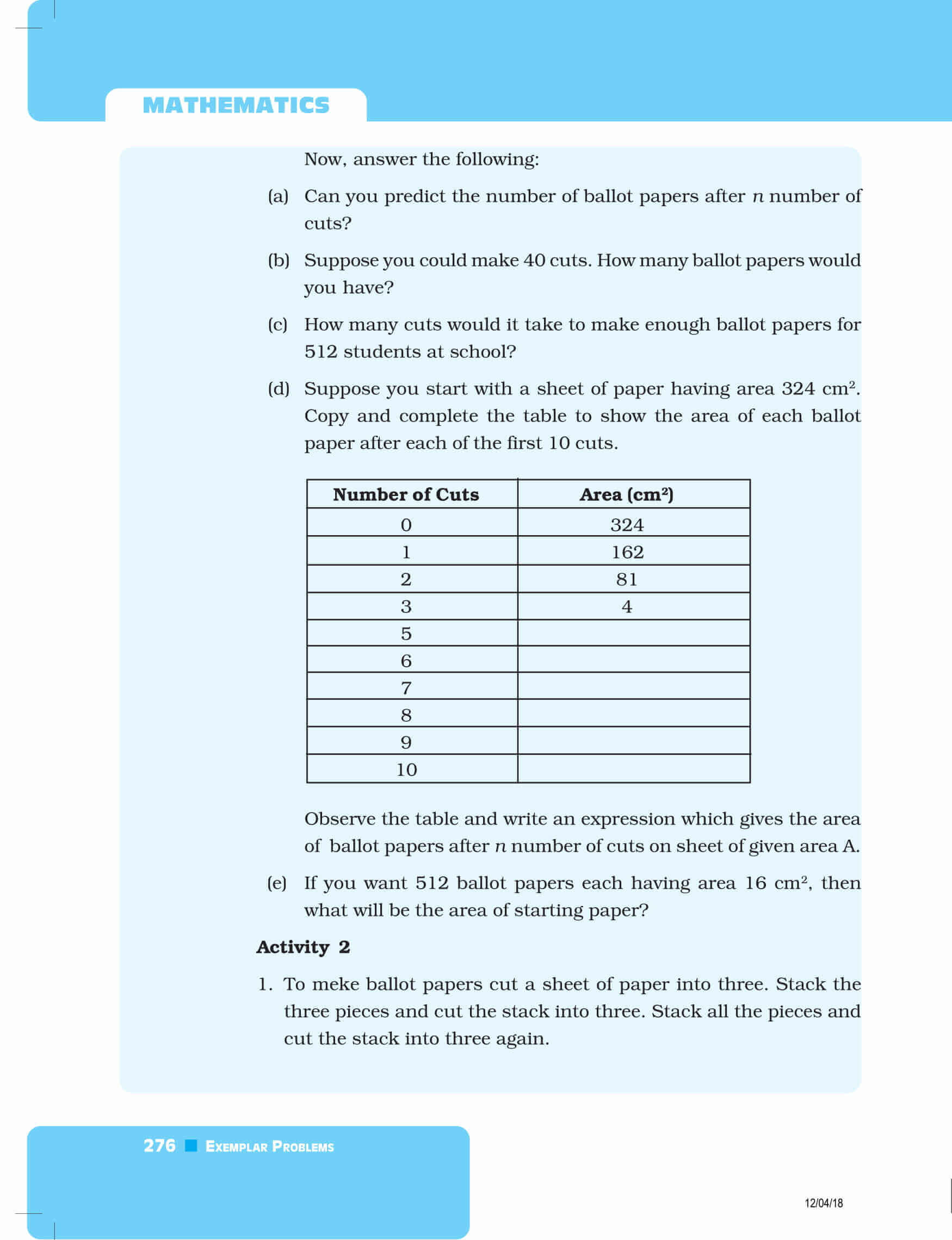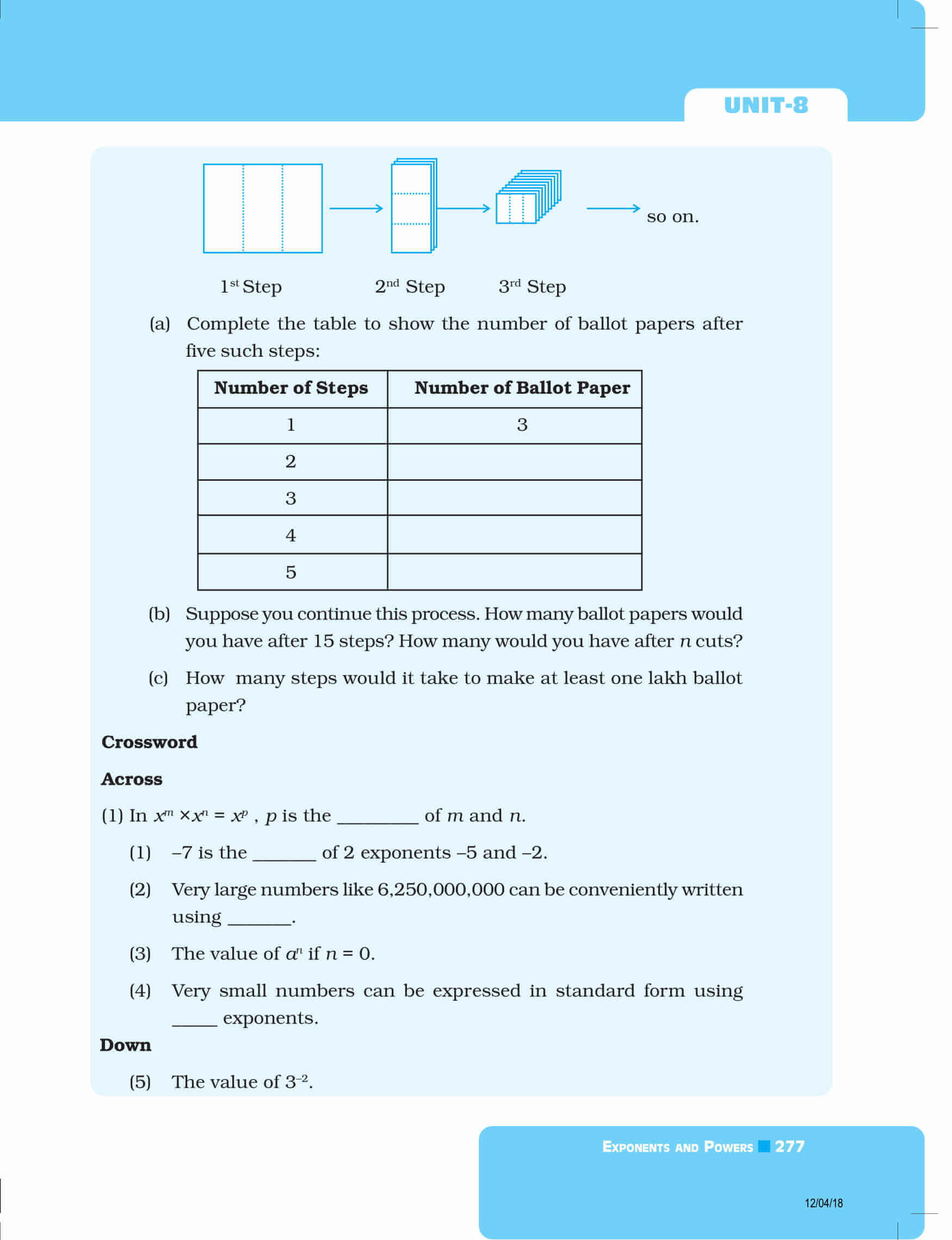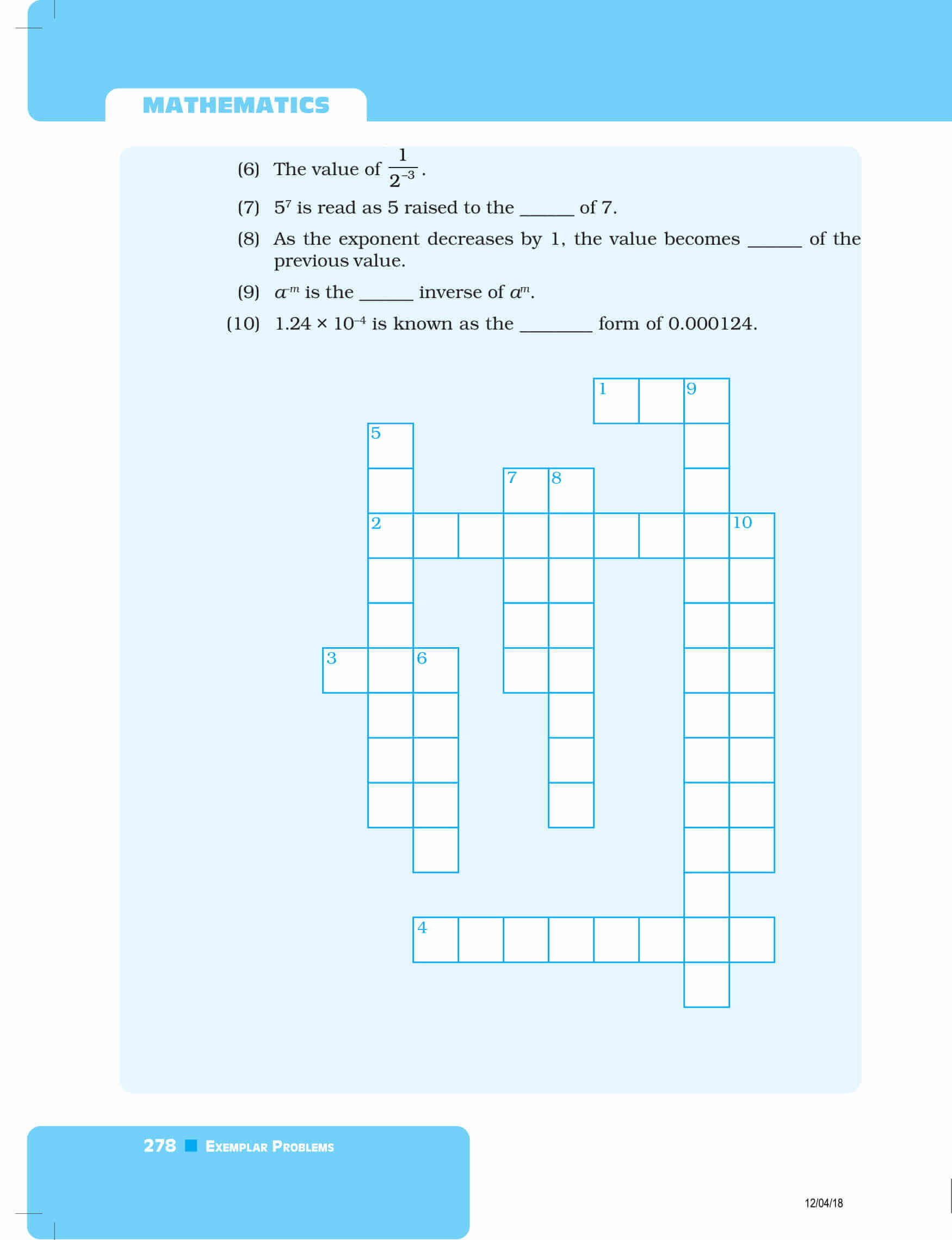Download exemplars solutions for all chapters of Maths covered in 8th class by clicking here. Also, download BYJU’S-The Learning App and get personalized videos, explaining the concepts of exponents and powers and many maths-related topics, experiencing a new way of learning to understand the concepts easily.

Also Check